• ## 利用BP神经网络进行函数拟合

万次阅读 多人点赞 2020-01-11 19:27:48
利用BP神经网络进行函数拟合摘要关键词问题描述算法设计结果分析与讨论结论源代码 摘要 数据拟合是在假设模型结构已知的条件下最优确定模型中未知参数使预测值与数据吻合度最高，本文选取线性项加激活函数组成一个非...


利用BP神经网络进行函数拟合
摘要关键词问题描述算法设计结果分析与讨论结论Python源代码

摘要
数据拟合是在假设模型结构已知的条件下最优确定模型中未知参数使预测值与数据吻合度最高，本文选取线性项加激活函数组成一个非线性模型，利用神经网络算法最优确定模型中的未知参数，利用随机搜索的方式确定函数模型，从而达到很好的拟合效果
关键词
BP神经网络 随机搜索 随机重启 参数优化 数据拟合 RELU
问题描述
数据拟合问题普遍存在，拟合问题本质上是在假设模型结构已知的条件下最优确定模型中未知参数以使得模型预测与数据的吻合度最高，即数据拟合问题往往描述为一个参数优化问题。 复杂工业过程对于模型精度的要求，线性模型往往不能满足。因此模型结构假设为非线性更为合理。此处我们假设模型结构为：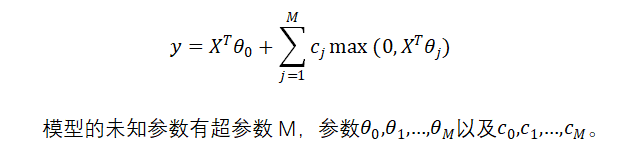算法设计
BP神经网络是一种按照误差逆向传播训练的多层前馈神经网络。神经网络理论上可以拟合任意曲线，这一点已经得到了严格的数学证明。
三层神经网络模型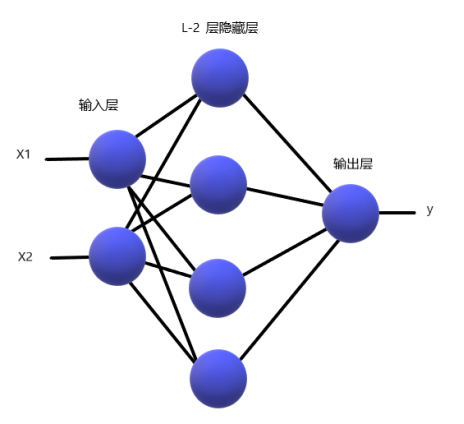通常一个多层神经网络由L层构成，其中有1层输出层，1层输入层，L-2层隐藏层。输入层是神经网络的第一层，表示一列矩阵或多列矩阵的输入。输出层是神经网络的最后一层，表示网络的输出结果，通常是一列矩阵。隐藏层有L-2层，表示每一层神经元通过前向传播算法计算的结果矩阵。 在本程序中，采用3层神经网络。输入矩阵X=[X1,X2]，题目中给出的模型需要对输入矩阵X进行增广，然后乘以参数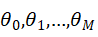，其实相当于神经网络中的偏置项bi，i=1，2。因此在本程序中无需对输入矩阵进行增广。wi，wi, i=1，2；bi，i=1，2分别是输入层与隐藏层之间，隐藏层与输出层之间的连接权重和偏置项。设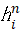，n=1，i=1，2，3，…，M，表示隐藏层的第i个神经元的输出。激活函数 本程序中采用的激活函数为RELU函数，即函数模型中的，目的是去除整个神经网络输出的线性化，使得整个神经网络模型呈非线性化。损失函数 本程序中采用的损失函数为MSE（均方误差），利用均方误差和激活函数的导数来更新权重和偏置。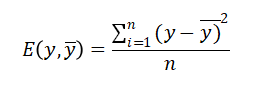前向传播算法是指依次向前计算相邻隐藏层之间的连接输出，直到模型的最终输出值。其特点作用于相邻层的两个神经元之间的计算，且前一层神经元的输出是后一层的神经元的输入。 设对于第n层的第i个神经元，有n-1层的M（M为超参数）个神经元与该神经元有突触相连，则第n层第i个神经元的输入为：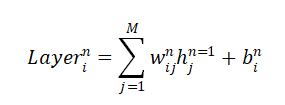第n层的第i个神经元的输出为：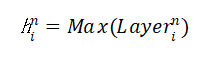反向传播算法 结合前向传播的两个式子，得到第n层第i个神经元的输入与第n-1层第i个神经元的输出的关系为：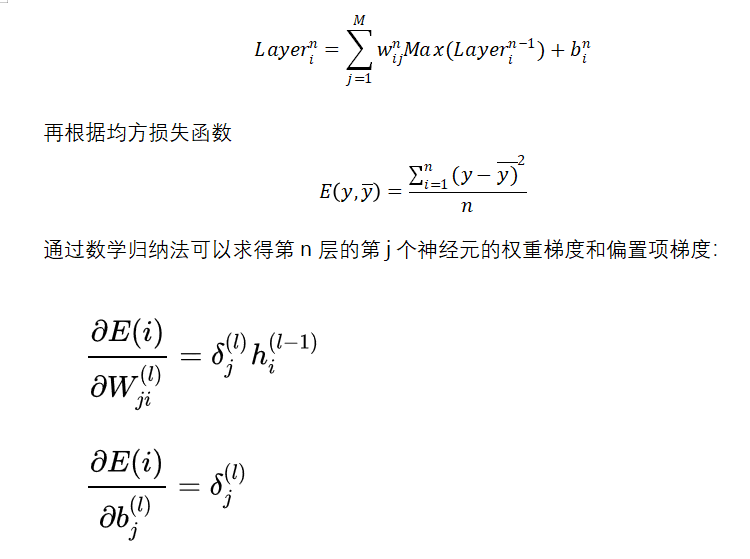梯度下降算法 得到每一个隐藏层的连接权重和偏置项的梯度后，利用学习率和梯度下降算法更新每一层的连接权重和偏置项：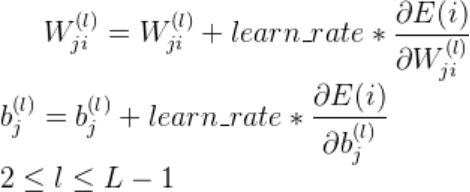学习率更新策略 在配合梯度下降优化的过程中，如果学习率设置过大，则容易导致模型过拟合；如果设置过小，会使得模型优化的速度变得很缓慢。为此加入衰减因子和学习次数来计算模型每次学习的学习率：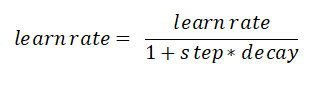归一化与反归一化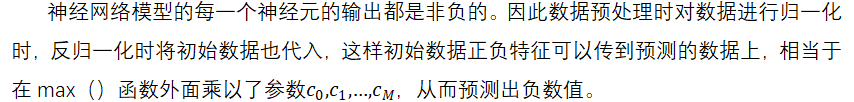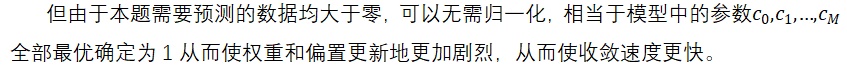随机重启算法 为了防止更新参数时陷入局部极小，采用随机重启的方式更换初始参数状态。超参数M的确定 本程序的超参数M的确定方法为随机搜索法，最终确定M的取值为20。编程环境和程序主要函数说明 全部内容均在JetBrains发布的PyCharm社区版（版本号2019.2.5）编程环境中完成，使用的语言为Python，解释器为Python3.8。
#产生数据，并将产生的数据方阵降维，方便计算处理 def init(inpit_n_row):
#激活函数 def relu(x):
#激活函数RELU的导数 def d_relu(x):
#归一化数据 def normalize(data):
#反归一化数据 def d_normalize(norm_data, data):
#前向传播算法 def prediction(l0, W, B):
#反向传播算法：根据损失函数优化各个隐藏层的权重 def optimizer(Layers, W, B, y, learn_rate):
#训练BP神经网络 def train(X, y, W, B, method, learn_rate):
#拟合曲面可视化 def visualize(y, inpit_n_row, input_n_col, hidden_n_1):
#为了解决陷入局部极小值，随机重启 def random_restart():
#main（）函数 if name == ‘main’:
结果分析与讨论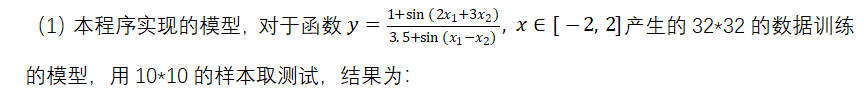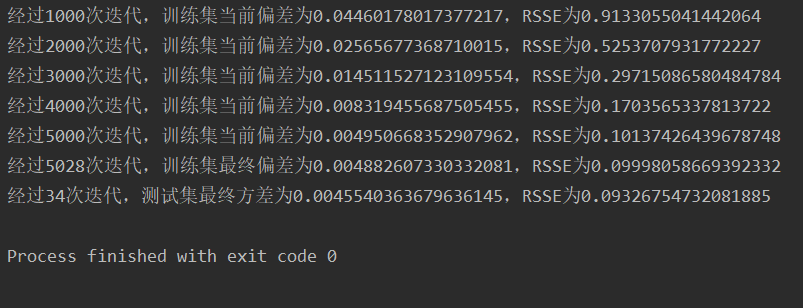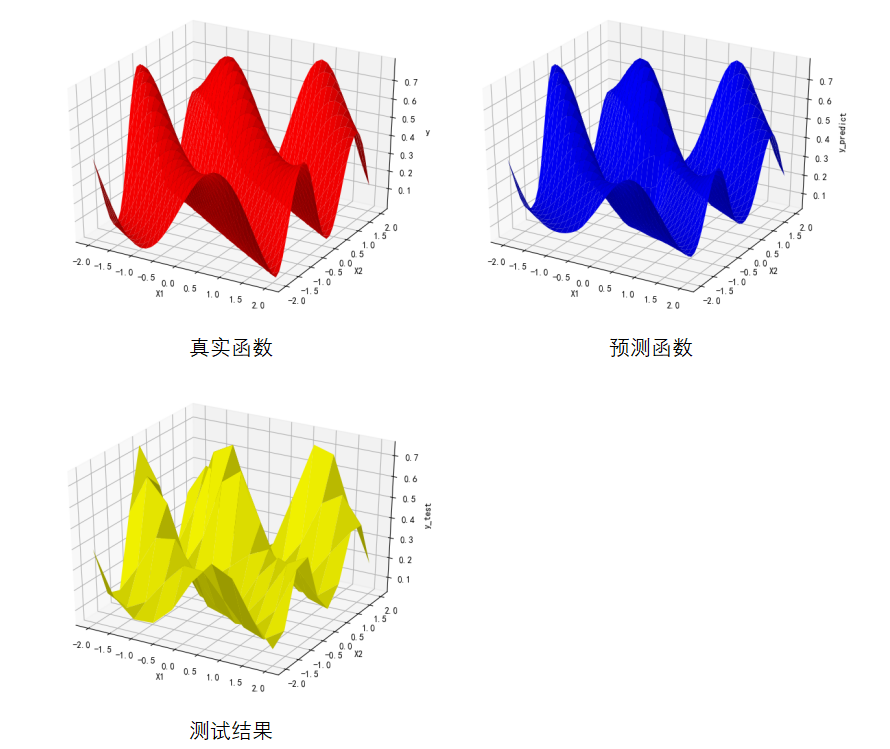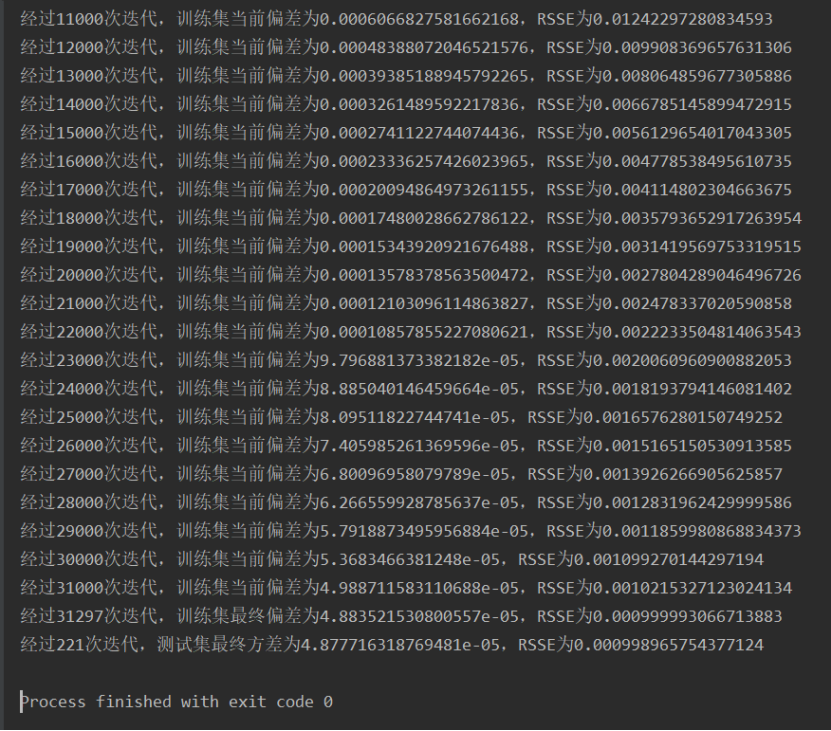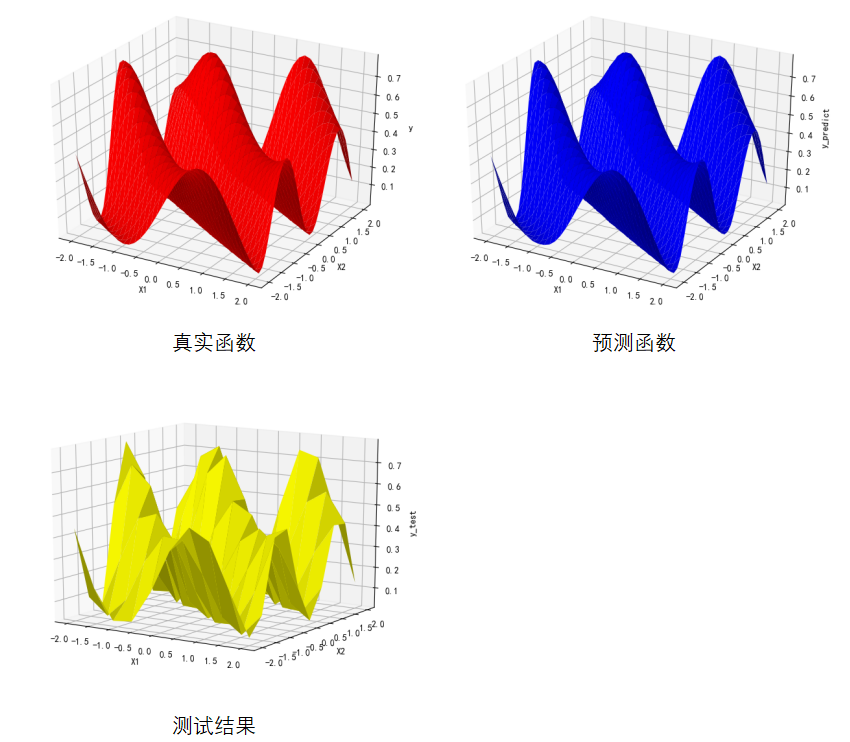对于3232的训练集，需要几千次的迭代，即可使模型的RSSE值降到0.1以下，需要10000-32000次左右的迭代，即可使模型的RSSE值降到0.001以下；对于1010的测试集，需要30-90次的迭代即可使其RSSE值降到0.1以下，需要200-300次左右的迭代，即可使其的RSSE值降到0.001以下。 (2)抗噪测试 加入高斯白噪声：a = (np.random.random() - 0.5)/5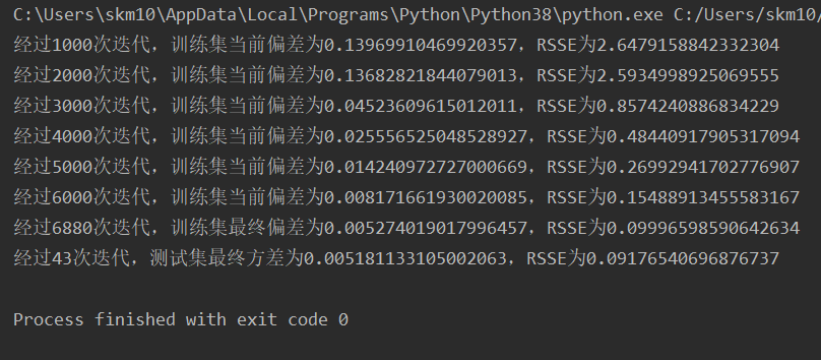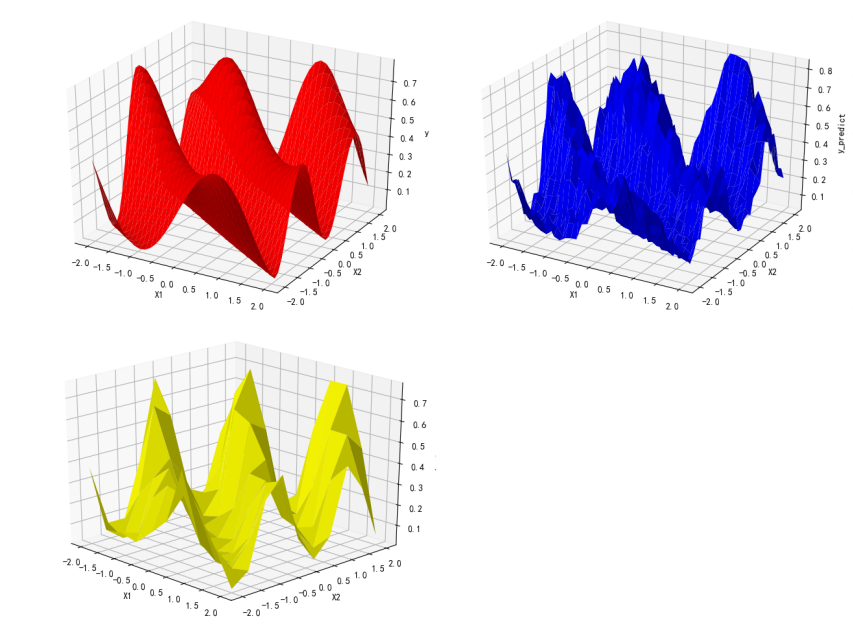加入野值： b = （np.random.randint(0, 9) - 0.5）/ 9 post = np.random.randint(0, inpit_n_row - 1) y[post] += b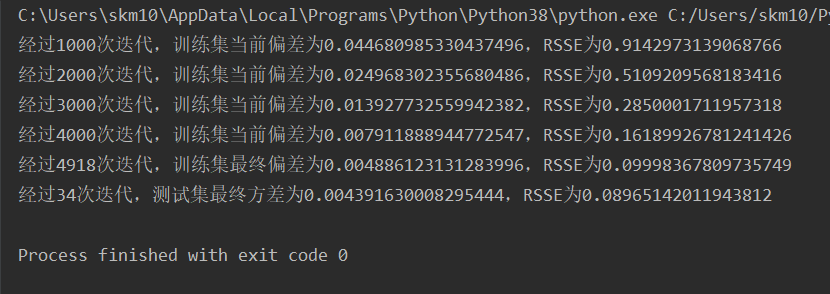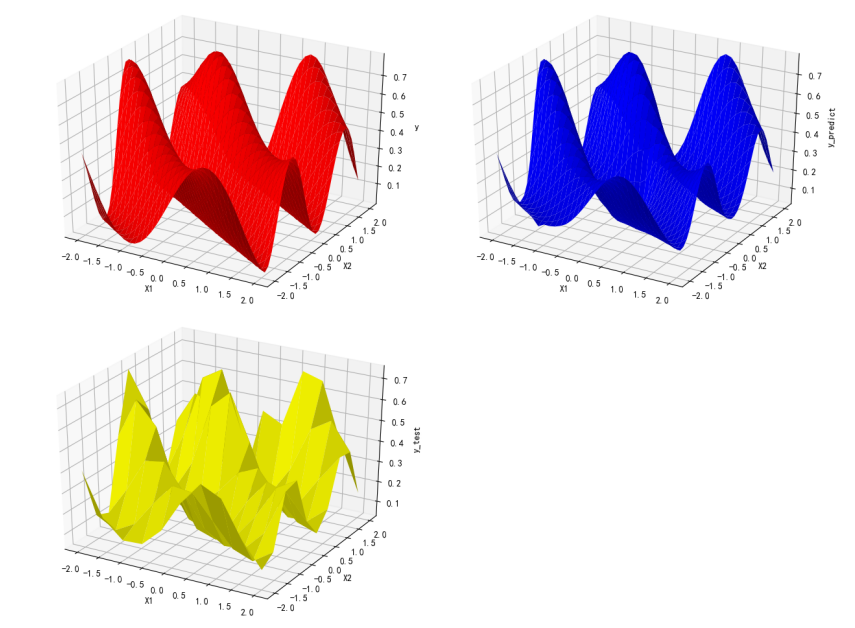由此可见，模型的抗噪能力良好。
结论
神经网络具有实现任何复杂非线性映射的功能。BP神经网络的的学习速度慢，因其本质上是梯度下降法，必然会出现锯齿现象。随机重启、模拟退火这些算法可以有效地解决陷入局部极值的问题。
Python源代码
import numpy as np
import matplotlib.pyplot as plt
from mpl_toolkits.mplot3d import Axes3D

plt.rcParams['axes.unicode_minus'] = False
plt.rcParams['font.sans-serif'] = ['SimHei']

#产生数据，并将产生的数据方阵降维，方便计算处理
def init(inpit_n_row):
X1 = np.linspace(-2, 2, int(inpit_n_row**0.5))
X2 = np.linspace(-2, 2, int(inpit_n_row**0.5))
X1, X2 = np.meshgrid(X1, X2)
X1 = X1.reshape(-1)
X2 = X2.reshape(-1)
x, y = [], []
for i in range(inpit_n_row):
# 增加高斯白噪声
#a = np.random.random() - 0.5
#a /= 5
x.append([X1[i], X2[i]])
Y = (1 + np.sin(2 * X1[i] + 3 * X2[i])) / (3.5 + np.sin(X1[i] - X2[i])) # + a
y.append(Y)
# 增加野值
'''b = np.random.randint(0, 9) - 0.5
b /= 9
post = np.random.randint(0, inpit_n_row - 1)
y[post] += b'''
x = np.array(x)
y = np.array([y]).T
return x,y

# 激活函数
def relu(x):
return np.maximum(0,x)

# 激活函数RELU的导数
def d_relu(x):
x[x<0] = 0
return x

# 归一化数据
def normalize(data):
data_min, data_max = data.min(), data.max()
data = (data - data_min) / (data_max - data_min)
return data

# 反归一化数据
def d_normalize(norm_data, data):
data_min, data_max = data.min(), data.max()
return norm_data * (data_max - data_min) + data_min

# 计算损失函数：平方损失函数
inpit_n_row = len(y)
return np.sum(1 / inpit_n_row * np.square(y_ - y))

y_ = np.mean(y)
return np.sum(np.square(y_predict - y))/np.sum(np.square(y-y_))

# 前向传播算法
def prediction(l0, W, B):
Layers = [l0]
for i in range(len(W)):
Layers.append(relu(x=Layers[-1].dot(W[i]) + B[i]))
return Layers

# 反向传播算法：根据损失函数优化各个隐藏层的权重
def optimizer(Layers, W, B, y, learn_rate):
l_error_arr = [(y - Layers[-1]) * d_relu(x=Layers[-1])]
for i in range(len(W) - 1, 0, -1):
l_error_arr.append(l_error_arr[-1].dot(W[i].T) * d_relu(x=Layers[i]))
j = 0  # l_delta_arr = [err3, err2, err1]
for i in range(len(W) - 1, -1, -1):
W[i] += learn_rate * Layers[i].T.dot(l_error_arr[j])  # W3 += h2 * err3
B[i] += learn_rate * l_error_arr[j]  # B3 += err3
j += 1

# 训练BP神经网络
def train(X, y, W, B, method, learn_rate):
#norm_X, norm_y = normalize(data=X), normalize(data=y)  # 归一化处理
norm_X = X
norm_y = y  # 由于测试模型无负值，无需归一化处理，可极大提高效率

#加了噪声之后，需降低精度要求，降低至0.1即可
end_loss = 0.001
step_arr, loss_arr, rsse_arr = [], [], []
step = 1
if method == '训练集':
error = '偏差'
else:
error = '方差'
while True:
Layers = prediction(l0=norm_X, W=W, B=B)
learn_rate_decay = learn_rate # * 1.0 / (1 + step * 0.001)
optimizer(Layers=Layers, W=W, B=B, y=y, learn_rate=learn_rate_decay)
if step % 1000 == 0:
step_arr.append(step)
loss_arr.append(cur_loss)
# prediction_ys = d_normalize(norm_data=Layers[-1], data=y)  # 反归一化结果
return 0, Layers[-1]
print('此初始权重可能导致算法陷入局部极值，故随机重启，重新迭代')
return -1, []
step += 1

# 拟合曲面可视化
def visualize(y, inpit_n_row, input_n_col, hidden_n_1):
z = []
for i in y:
for j in i:
z.append(j)
z = np.array(z).reshape(int(inpit_n_row**0.5), int(inpit_n_row**0.5))
# 代入训练好的模型测试
flag = -1
while flag == -1:
np.random.seed(random_restart())
# 产生测试集
test_n_row = 100
x_test, y_test = init(test_n_row)
W1 = np.random.randn(input_n_col, hidden_n_1) / np.sqrt(test_n_row)
b1 = np.zeros((test_n_row, hidden_n_1))
# 输出层权重矩阵
W2 = np.random.randn(hidden_n_1, 1) / np.sqrt(test_n_row)
b2 = np.zeros((test_n_row, 1))
w, b = [W1, W2], [b1, b2]
flag, y_test = train(x_test, y_test, w, b, '测试集', learn_rate=0.15)
z_test = []
for i in y_test:
for j in i:
z_test.append(j)
z_test = np.array(z_test).reshape(int(test_n_row ** 0.5), int(test_n_row ** 0.5))
# 拟合曲面可视化
X1 = np.linspace(-2, 2, int(inpit_n_row**0.5))
X2 = np.linspace(-2, 2, int(inpit_n_row**0.5))
X1, X2 = np.meshgrid(X1, X2)
Z = (1 + np.sin(2 * X1 + 3 * X2)) / (3.5 + np.sin(X1 - X2))
fig = plt.figure()
ax = Axes3D(fig)
# 画出真实函数
ax.set_xlabel('X1')
ax.set_ylabel('X2')
ax.set_zlabel('y')
ax.plot_surface(X1, X2, Z, color='red')
# 画出预测函数
fig = plt.figure()
ax = Axes3D(fig)
ax.set_xlabel('X1')
ax.set_ylabel('X2')
ax.set_zlabel('y_predict')
ax.plot_surface(X1, X2, z, color='blue')
# 画出测试结果
X1 = np.linspace(-2, 2, int(test_n_row ** 0.5))
X2 = np.linspace(-2, 2, int(test_n_row ** 0.5))
X1, X2 = np.meshgrid(X1, X2)
fig = plt.figure()
ax = Axes3D(fig)
ax.set_xlabel('X1')
ax.set_ylabel('X2')
ax.set_zlabel('y_test')
ax.plot_surface(X1, X2, z_test, color='yellow')
plt.show()

# 为了解决陷入局部极小值，随机重启
def random_restart():
return np.random.randint(0, 23768)

if __name__ == '__main__':
# 样本点个数
inpit_n_row = 1024
# 输入维度
input_n_col = 2
# 神经元个数
hidden_n_1 = 20
flag = -1
while flag == -1:
np.random.seed(random_restart())
x, y = init(inpit_n_row)
# 第一个隐藏层权重矩阵
W1 = np.random.randn(input_n_col, hidden_n_1) / np.sqrt(inpit_n_row)
b1 = np.zeros((inpit_n_row, hidden_n_1))
# 输出层权重矩阵
W2 = np.random.randn(hidden_n_1, 1) / np.sqrt(inpit_n_row)
b2 = np.zeros((inpit_n_row, 1))
W, B = [W1, W2], [b1, b2]
flag, y = train(x, y, W, B, '训练集', learn_rate=0.001)  # 开始训练BP神经网络
visualize(y, inpit_n_row, input_n_col, hidden_n_1)

展开全文人工智能 机器学习 算法 python
• ## 利用BP神经网络逼近函数(Python)

千次阅读 多人点赞 2019-04-19 22:00:37
前面我们讲了关于张量、张量运算、激活函数、代价函数相关的一系列文章，本篇将使用Python 3从头实现一个神经网络，用来逼近函数，有理论证明，神经网络可以逼近任何函数。本篇作为例子，我们使用神经网络逼近f(x)=...
回顾上一篇：深刻理解机器学习的: 目标函数，损失函数和代价函数
引言
前面我们讲了关于张量、张量运算、激活函数、代价函数相关的一系列文章，本篇将使用Python 3从头实现一个神经网络，用来逼近函数，有理论证明，神经网络可以逼近任何函数。本篇作为例子，我们使用神经网络逼近

f

(

x

)

=

s

i

n

(

x

)

f(x)=sin(x)

函数，让大家初步领略神经网络的魔力。 为了确保你能正确运行本章代码，确保你有：PyCharm IDE，安装好Python虚拟机，安装好Tensorflow以及相关Python包。
文章目录：
引出问题定义神经网络结构产生输入数据以及数据的组织选择和实现激活函数计算前馈网络的输出选择和实现损失函数计算BP网络梯度和更新权重实现训练网络运行自编写Python代码和展示结果用TensorFlow实现以及结果对比拓展本例小结
引出问题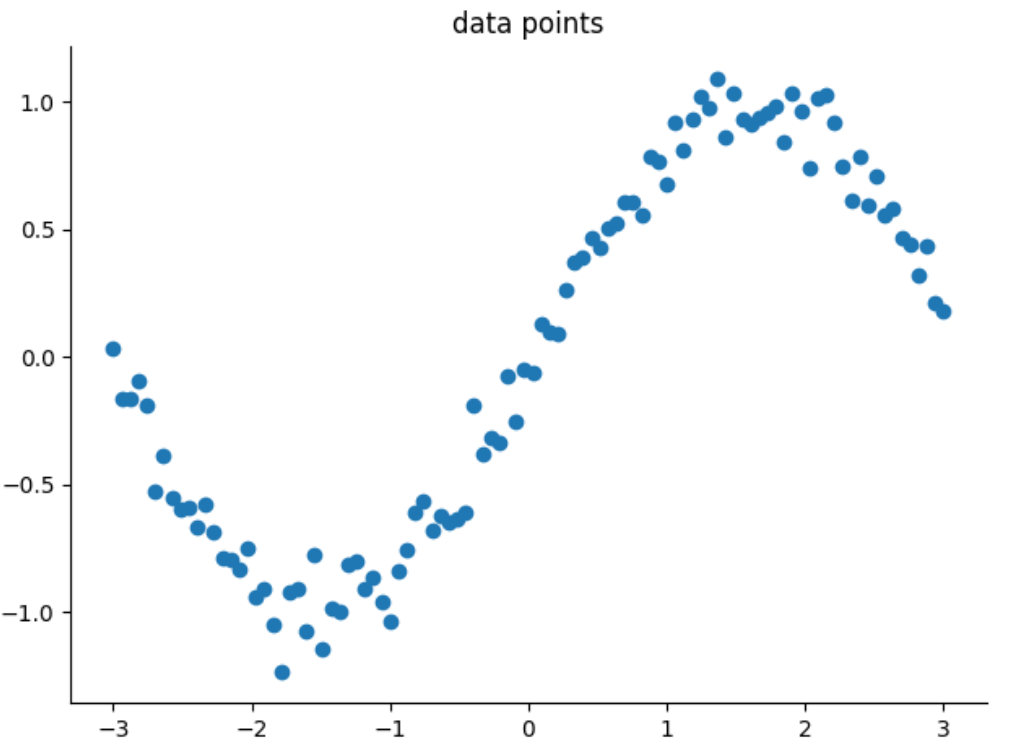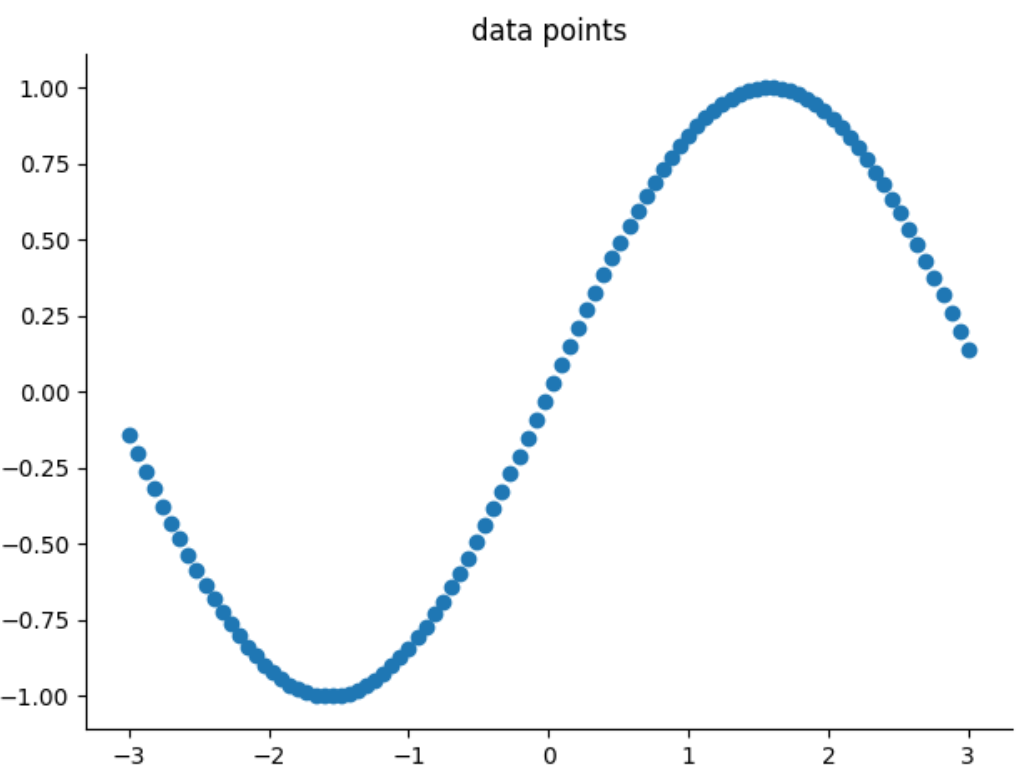以上两张图，一张是加了噪点点数据集，一张是没有加点，对于神经网络来说，它并不管你是正弦或者余弦，它只知道使用神经网络的方法可以找到规律或者模型，以便你输入新点x值，它可以告诉你对应点y值。
定义神经网络结构
首先面临的第一个问题，使用什么样的神经网络结构？神经网络结构怎么定义？很显然，只需要使用浅层神经网络结构即可，是个简单线性回归问题，这里我们使用两层，一个隐藏层，一个输出层的网络来实现模型的训练，网络示意图如下：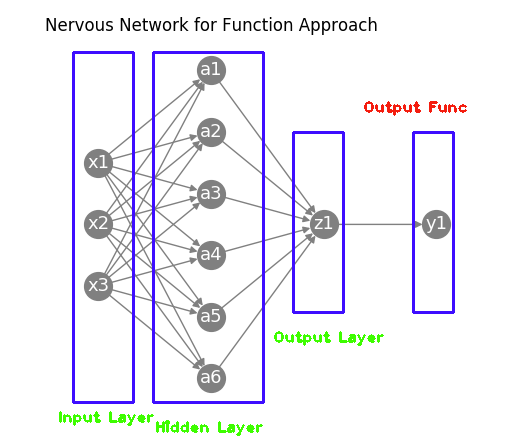x

1

,

x

2

,

x

3

x1,x2,x3

这层属于输入层；

a

1

～

a

10

a1～a10

是隐藏层，图里面只画了6个，实际上有数据点个数相同多个神经元，这层的神经元要使用激活函数；

z

1

z1

是输出层，1个神经元，这个神经元里不用激活函数，这层的输出就是结果。输入层和隐藏层之间有权重W1和偏置B1，隐藏层与输出层之间有权重W2和和偏置B2。
我们定义一个Python类 ApproachNetwork 来实现，根据网络结构定义，可以在__init__里定义：
def __init__(self, hidden_size=100, output_size=1):
self.params = {'W1': np.random.random((1, hidden_size)),
'B1': np.zeros(hidden_size),
'W2': np.random.random((hidden_size, output_size)),
'B2': np.zeros(output_size)}

产生输入数据以及数据的组织
怎么把数据输入到神经网络？以什么形式呢？这是需要好好思考的问题。 前面在这篇文章了解机器学习(深度学习)的几个特点里说过，输入数据的维度以及含义，这里我们把数据组织形状为 (samples, features)这样的形式, samples代表数据点个数，features代表每个数据点的特征数，在这里因为只有一个自变量，特征数为1，如果我们产生100个数据点，那么数据的形状是(100,1), 我们可以一次行把这个矩阵数据输入神经网络，即上面的输入层神经元个数为100. 为了产生这样的训练数据，可以利用文章np.random用法里面的Numpy方法，在类定义里增加产生数据的函数generate_data，函数的参数含义代码里有解释，这个函数具有一定的通用性，可以为不同函数产生训练数据集：
@staticmethod
def generate_data(fun, is_noise=True, axis=np.array([-1, 1, 100])):
"""
产生数据集
:param fun: 这个是你希望逼近的函数功能定义，在外面定义一个函数功能方法，把功能方法名传入即可
:param is_noise: 是否需要加上噪点，True是加，False表示不加
:param axis: 这个是产生数据的起点，终点，以及产生多少个数据
:return: 返回数据的x, y
"""
np.random.seed(0)
x = np.linspace(axis, axis, axis)[:, np.newaxis]
x_size = x.size
y = np.zeros((x_size, 1))
if is_noise:
noise = np.random.normal(0, 0.1, x_size)
else:
noise = None

for i in range(x_size):
if is_noise:
y[i] = fun(x[i]) + noise[i]
else:
y[i] = fun(x[i])

return x, y

对于函数

f

(

x

)

=

x

2

f(x)=x^2

调用上面的方法产生数据并把它显示出来，如下：
# 逼近函数 f(x)=sin(x)
def fun_sin(x0):
return math.sin(x0)

x, y = network.generate_data(fun_sin, False, axis=np.array([-3, 3, 100]))
ax = plt.gca()
ax.set_title('data points')
ax.spines['right'].set_color('none')
ax.spines['top'].set_color('none')
plt.scatter(x, y)
plt.show()

图形如下：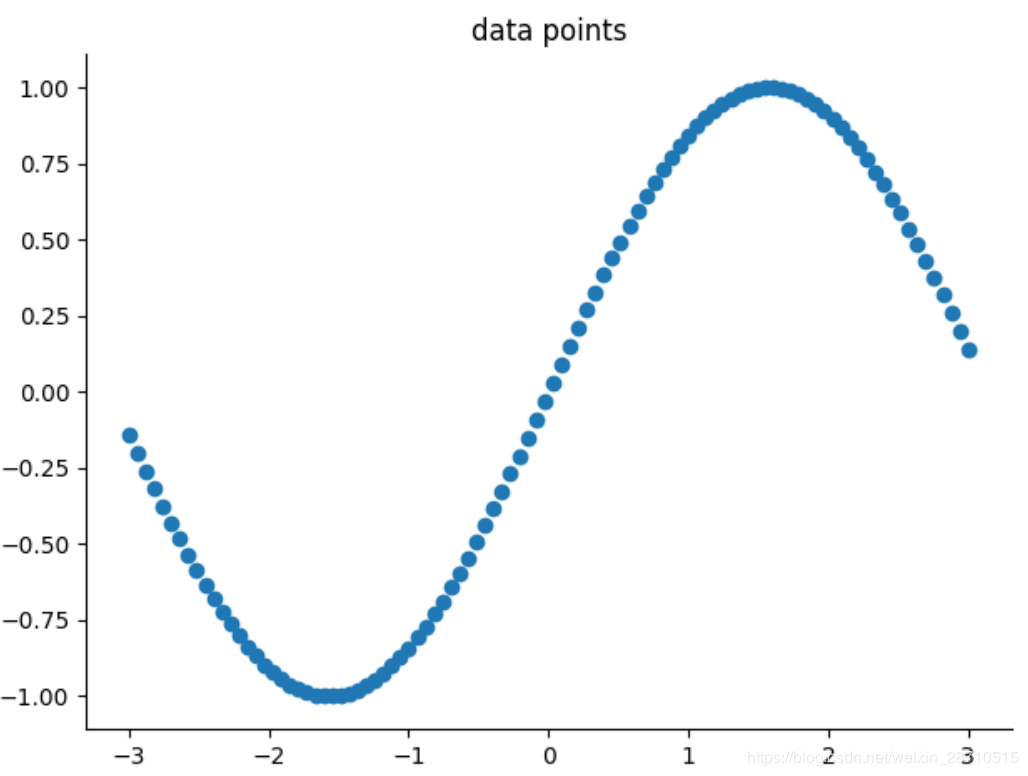选择和实现激活函数
激活函数选择

s

i

g

m

o

i

d

sigmoid

或

r

e

l

u

relu

都可以，这里选择

s

i

g

m

o

i

d

sigmoid

，Python实现如下，注意还需要实现其导数以便在BP反向传播时使用，导数推导过程这里不讲，自行百度：
@staticmethod
def sigmoid(x_):
return 1 / (1 + np.exp(-x_))

return (1.0 - self.sigmoid(x_)) * self.sigmoid(x_)

计算前馈网络的输出
从输入到输出结果计算，隐藏层用激活函数

s

i

g

m

o

i

d

sigmoid

，输出层不用激活函数，用Python实现predict方法，如下：
def predict(self, x_):
W1, W2 = self.params['W1'], self.params['W2']
b1, b2 = self.params['B1'], self.params['B2']

a1 = np.dot(x_, W1) + b1
z1 = self.sigmoid(a1)
a2 = np.dot(z1, W2) + b2

return a2

选择和实现损失函数
对于本例，因为是对比输出值的偏差，所以选择均方差MSE作为损失函数或者叫优化函数，定义

l

o

s

s

loss

函数定义实现。
def loss(self, x_, t):
y_ = self.predict(x_)
return y_, np.mean((t - y_) ** 2)

计算BP网络梯度和更新权重
神经网络是根据计算出来的

l

o

s

s

loss

不断调整权重和偏置，以便不断减少

l

o

s

s

loss

,达到逼近函数的目的。Python实现代码如下：
def gradient(self, x, t):
W1, W2 = self.params['W1'], self.params['W2']
b1, b2 = self.params['B1'], self.params['B2']

batch_num = x.shape

# forward
a1 = np.dot(x, W1) + b1
z1 = self.sigmoid(a1)
a2 = np.dot(z1, W2) + b2

# backward
dy = (a2 - t) / batch_num

dz1 = np.dot(dy, W2.T)

# 根据上述计算的梯度，结合学习率，更新权重和偏置
for key in ('W1', 'B1', 'W2', 'B2'):

实现训练网络
有了上面的步骤，就可以定义训练主函数，主要代码：
def train_with_own(self, x_, y_, max_steps=100):
for k in range(max_steps):
for key in ('W1', 'B1', 'W2', 'B2'):
pred, loss = network.loss(x_, y_)

if k % 150 == 0:
# 动态绘制结果图，你可以看到训练过程如何慢慢的拟合数据点
plt.cla()
plt.scatter(x, y)
plt.plot(x, pred, 'r-', lw=5)
plt.text(0.5, 0, 'Loss=%.4f' % abs(loss), fontdict={'size': 20, 'color': 'red'})
plt.pause(0.1)

# 关闭动态绘制模式
plt.ioff()
plt.show()

运行自编写Python代码和展示结果
编写运行主函数，其中实例化类ApproachNetwork，调用产生数据和训练方法即可完成。
if __name__ == '__main__':
network = ApproachNetwork()
x, y = network.generate_data(fun_sin, False, axis=np.array([-3, 3, 100]))
# x, y = network.generate_data(fun_xx)

# 使用 自编代码 训练
network.train_with_own(x, y, 3500)

结果展示 以下图形是在设定最大循环步骤为3500，学习率为0.05下输出的，本例还采用了动态输出图形的办法，每150步，刷新输出当前图形，你可以直观看到训练过程中函数逼近的过程，本例是在PyCharm中开发、测试和运行。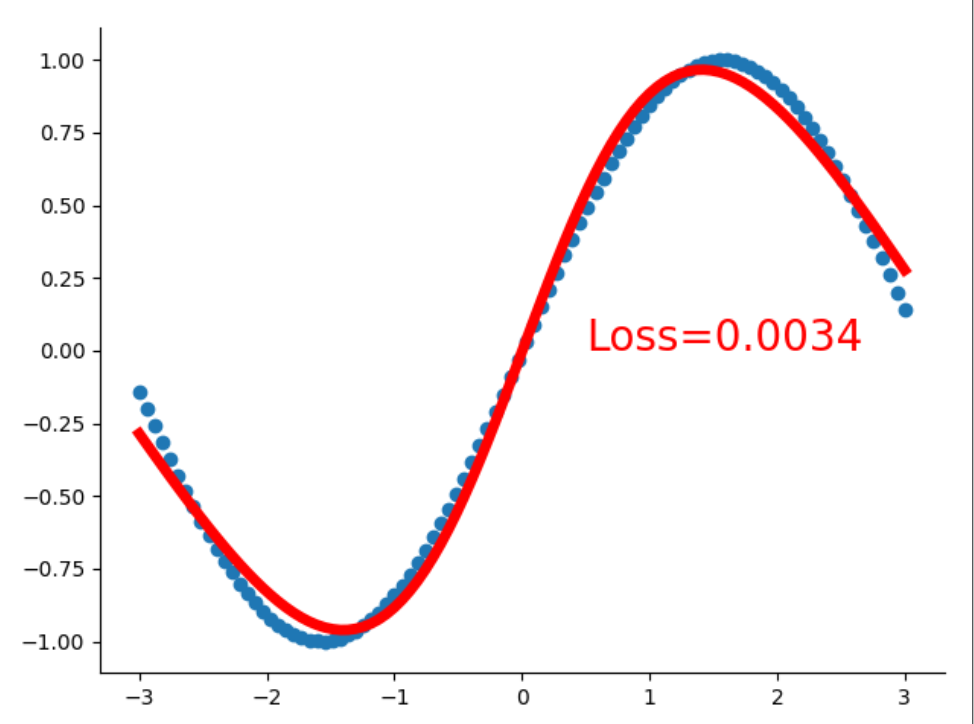用TensorFlow实现以及结果对比
函数逼近的问题，也可以使用Tensorflow这种深度学习框架来实现，在本例中在ApproachNetwork类中定义一个方法 train_with_tf，代码如下，解释在代码中
 @staticmethod
def train_with_tf(x, y):
tf_x = tf.placeholder(tf.float32, x.shape)  # input x
tf_y = tf.placeholder(tf.float32, y.shape)  # input y

# 神经网络定义, 激活函数使用 ReLU 或 sigmoid 都可以
# l1 = tf.layers.dense(tf_x, 10, tf.nn.relu)  # hidden layer
l1 = tf.layers.dense(tf_x, 10, tf.nn.sigmoid)  # hidden layer
output = tf.layers.dense(l1, 1)  # output layer

# 损失函数和优化器设置
loss = tf.losses.mean_squared_error(tf_y, output)  # compute cost

# 初始化
sess = tf.Session()
sess.run(tf.global_variables_initializer())

# 设置动态绘制，不是训练完了才绘制结果
plt.ion()

for step in range(1500):
_, l, pred = sess.run([train_op, loss, output], {tf_x: x, tf_y: y})
if step % 100 == 0:
# 动态绘制结果图，你可以看到训练过程如何慢慢的拟合数据点
plt.cla()
plt.scatter(x, y)
plt.plot(x, pred, 'r-', lw=5)
plt.text(0.5, 0, 'Loss=%.4f' % l, fontdict={'size': 20, 'color': 'red'})
plt.pause(0.1)

# 关闭动态绘制模式
plt.ioff()
plt.show()

可以看出，使用Tensorflow框架代码简洁，步骤清楚。但是自己用Python动手开发神经网络能使你清楚各个环节，对你提高能力和水平是大有裨益的。 在同样的条件设置下，逼近的结果如下：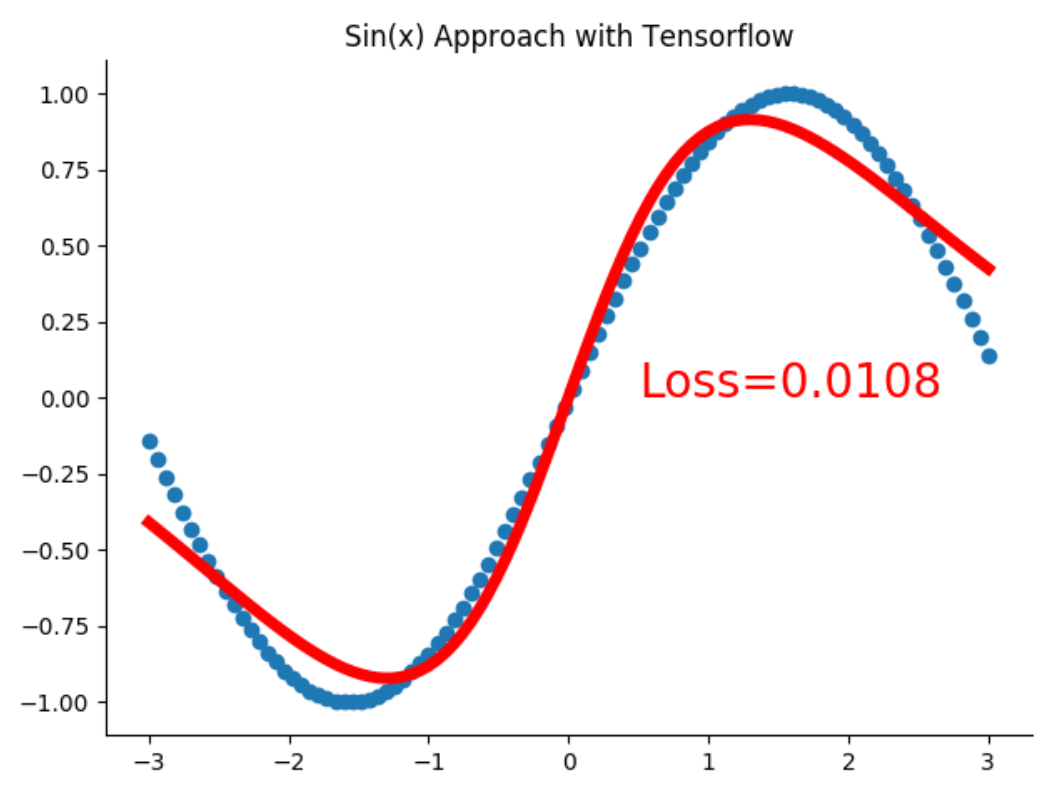比较起来，自己编写代码精度似乎还要高些！Cheers!
扩展本例
这个类不仅可以逼近正弦函数，还可以逼近你喜欢试的函数，例如我想让它逼近

f

(

x

)

=

x

2

f(x) = x^2

，先定义该函数，然后在生成数据参数中传递函数名进去，第三个参数根据需要修改一下，保证你的数据集能够完整的表现改函数的形状特征。在训练过程中，其它参数可以适当调整以下，如学习率，最大迭代次数等，这种可以把函数作为参数传递的做法，也是Python作为动态语言的一个优势所在，自己可以去体会：
# 逼近函数 f(x)=x**2
def fun_xx(x0):
return np.power(x0, 2)

x, y = network.generate_data(fun_xx)

小结
读者可能的疑惑是，BP反馈网络计算梯度不太明白，继续阅读 用计算图理解和计算BP神经网络的梯度。
参考文献
利用BP神经网络逼近函数——Python实现 机器学习与神经网络（四）：BP神经网络的介绍和Python代码实现 一个 11 行 Python 代码实现的神经网络
展开全文Python
• ## 神经网络Loss损失函数总结

万次阅读 多人点赞 2017-06-25 14:17:21
这篇博文总结一下损失函数，这里重点总结一下常用的损失函数。 softamx cross entropy loss softmax 交叉熵损失函数是我们...其中，t 和 y 分别表示神经网络的目标标签和输出， yjy_j 表示softmax损失函数： yj=s
这篇博文总结一下损失函数，这里重点总结一下常用的损失函数。  损失函数有很多，不得不说有很多人已经专门对比过了，比如ArXiv上面的这篇就很不错：  (https://arxiv.org/pdf/1702.05659.pdf)  (http://christopher5106.github.io/deep/learning/2016/09/16/about-loss-functions-multinomial-logistic-logarithm-cross-entropy-square-errors-euclidian-absolute-frobenius-hinge.html)
softamx cross entropy loss
softmax 交叉熵损失函数是我们常用的一种损失函数，其公式如下：

E(t,y)=−∑jtjlogyj

E(t, y)=-\sum_j t_j \log y_j
其中，
t 和
y 分别表示神经网络的目标标签和输出，

yj
$y_j$ 表示softmax损失函数：

yj=softmax(zj)=ezj∑jezj

y_j=softmax(z_j)=\frac{e^{z_j}}{\sum_j e^{z_j}}

需要注意的一点就是这个公式需要输入没有经过缩放变换的logits，还有就是使用本目标损失函数的时候不要在网络的最后一层使用softmax层或者激活函数，会导致结果不正确。

Categorical Crossentropy
交叉熵损失函数是也是常用的一种损失函数，它表示预测值y与目标值t之间的距离。主要应用在互相排斥的分类任务中，公式为：

H(y,t)=Ht(y)=−∑itilogyi

H(y , t)=H_{t}(y)=-\sum_{i}t_i\log y_i

Binary Crossentropy
这个损失函数主要是用来计算预测值y与目标值t之间的sigmoid交叉熵，主要用来多分类任务中，但是这个分类任务不是互斥的，和上面的损失函数不同，这个对同一个输入可以输出多个标签。公式为：

y−y∗t+log(1+exp(−y))

y - y*t{\rm{ }} + {\rm{ }}log\left( {1{\rm{ }} + {\rm{ }}exp\left( { - y} \right)} \right)
为了防止溢出，我们进行如下变换：

max(y,0)−y∗t+log(1+exp(−abs(y)))

max\left( {y,{\rm{ }}0} \right){\rm{ }} - {\rm{ y}}*t{\rm{ }} + {\rm{ }}log\left( {1{\rm{ }} + {\rm{ }}exp\left( { - abs\left( y \right)} \right)} \right)

Weighted Crossentropy
主要用来计算神经元之间的权值的交叉熵损失函数，t表示目标标签，y表示输入的预测值。该损失函数和上一个损失函数很像，唯一一点不同的就是：

该损失函数允许对负误差或者正误差加权 来调整precision 和recall

一般的交叉损失函数为：

t∗−log(sigmoid(y))+(1−t)∗−log(1−sigmoid(y))

t* - log\left( {sigmoid\left( y \right)} \right){\rm{ }} + {\rm{ }}\left( {1 - t} \right){\rm{ }}* - log\left( {1 - sigmoid\left( y \right)} \right)
当我们乘上
pos_weight 之后的公式就变成：

t∗−log(sigmoid(y))∗pos_weight+(1−t)∗−log(1−sigmoid(y))

t* - log\left( {sigmoid\left( y \right)} \right)*pos\_weight{\rm{ }} + {\rm{ }}\left( {1 - t} \right){\rm{ }}* - log\left( {1 - sigmoid\left( y \right)} \right)
为了避免溢出，我们将公式变为：

(1−t)∗y+l∗(log(1+exp(−abs(y)))+max(−y,0))

\left( {1{\rm{ }} - {\rm{ t}}} \right){\rm{ }}*{\rm{ y }} + {\rm{ }}l{\rm{ }}*{\rm{ }}\left( {log\left( {1{\rm{ }} + {\rm{ }}exp\left( { - abs\left( y \right)} \right)} \right){\rm{ }} + {\rm{ }}max\left( { - y,{\rm{ }}0} \right)} \right)
其中，

l
$l$ 表示：
l=(1+(pos_weight−1)∗t)l{\rm{ }} = {\rm{ }}\left( {1{\rm{ }} + {\rm{ }}\left( {{\rm{pos\_weight }} - {\rm{ }}1} \right){\rm{ }}*{\rm{ t}}} \right)

Mean Square Loss
这个损失函数就很常见了，t 表示目标值，y 表示预测值输出。公式为：

MSE=1n∑i=1n(yi−ti)2

MSE = \frac{1}{n}\sum\limits_{i = 1}^n {{{({y_i} - {t_i})}^2}}

Hinge Loss
这个也是很常见的一个loss函数， t 表示目标值，y 表示预测值输出。公式为：

ℓ(y)=max(0,1−t∗y)

\ell (y) = \max (0,1 - t*y)

ROC AUC Score
使用 Wilcoxon-Mann-Whitney Statistic[详见] 统计量来逼近ROC曲线下面积。t 表示目标值，y 表示预测值输出。（这个目标损失函数目前我也理解的不是很好，如果有那位理解了可以给我留言指导一下）。  (http://tflearn.org/objectives/#roc-auc-score)
Weak Softmax Crossentropy 2d
这个目标损失函数使用弱交叉熵计算图像分割方面的损失，输入是一个2d形状的预测值y和目标值t 从而计算出分割的损失。 t 表示目标值，y 表示预测值输出。  （这个理解也不是很深，以后再写吧）  (http://tflearn.org/objectives/#weak-crossentropy-2d)
Contrastive Loss
对比损失函数。这个损失函数主要用在Siamese network中，参见论文(http://yann.lecun.com/exdb/publis/pdf/chopra-05.pdf)。d 表示目标值，y 表示预测值输出。

L=12N∑n=1Nyd2+(1−y)max(margin−d,0)2

L=\frac{1}{2N}\sum_{n=1}^Nyd^2+(1-y)max(margin-d,0)^2
其中

d
$d$ 表示两个样本特征的欧氏距离
d=||xi−xj||2d=||x_i - x_j||_2

: Yan, L., Dodier, R., Mozer, M. C., & Wolniewicz, R. (2003). Optimizing Classifier Performance via an Approximation to the Wilcoxon-Mann-Whitney Statistic.  : Sumit Chopra, Raia Hadsell and Yann LeCun (2005). Learning a Similarity Metric Discriminatively, with Application to Face Verification.
展开全文• 神经网络结构以及前向传播过程 损失函数和代价函数 反向传播 1 矩阵补充知识 11 矩阵求梯度 12 海塞矩阵 13 总结 2 矩阵乘积和对应元素相乘 3 反向传播原理四个基础等式 4 反向传播总结 41 单样本输入公式表 42 多...


文章目录
0. 什么是人工神经网络？1. 神经网络初探1.1 神经元模型1.2 神经元激活函数1.3 神经网络结构
2. 损失函数和代价函数3. 反向传播3.1 矩阵补充知识**3.1.1 求梯度矩阵****3.1.2 海塞矩阵****3.1.3 总结**
3.3 梯度下降法原理3.4 反向传播原理（四个基础等式）3.5 反向传播总结**3.5.1 单样本输入公式表****3.5.2 多样本输入公式表****3.5.3 关于超参数**

4. 是不是猫？4.1 辅助函数4.2 前向传播过程4.3 反向传播过程4.4 测试结果
5. 本文小结

订正与答疑：1. 具体解释一下公式1里面的“堆砌”是什么意思？2. 公式2写成矩阵形式为什么系数矩阵会有转置？自己没搞懂。3. 公式3能具体讲一下矩阵形式是怎么来的吗？4. 为什么会损失函数不用最小二乘法？5. 能不能顺便介绍一下Python环境的管理？6. 为什么w的初始化使用随机初始化，而b参数的初始化全部初始化为0？

本文着重讲述经典BP神经网络的数学推导过程，并辅助一个小例子。本文不会介绍机器学习库(比如sklearn, TensorFlow等)的使用。 欲了解卷积神经网络的内容，请参见我的另一篇博客一文搞定卷积神经网络——从原理到应用。

本文难免会有叙述不合理的地方，希望读者可以在评论区反馈。我会及时吸纳大家的意见，并在之后的chat里进行说明。
本文参考了一些资料，在此一并列出。
http://neuralnetworksanddeeplearning.com/chap2.htmlhttps://www.deeplearning.ai/ coursera对应课程视频讲义coursera华盛顿大学机器学习专项周志华《机器学习》李航《统计学习方法》张明淳《工程矩阵理论》

0. 什么是人工神经网络？
首先给出一个经典的定义：“神经网络是由具有适应性的简单单元组成的广泛并行互连网络，它的组织能够模拟生物神经系统对真实世界物体所作出的交互反应”[Kohonen, 1988]。
这种说法虽然很经典，但是对于初学者并不是很友好。比如我在刚开始学习的时候就把人工神经网络想象地很高端，以至于很长一段时间都不能理解为什么神经网络能够起作用。类比最小二乘法线性回归问题，在求解数据拟合直线的时候，我们是采用某种方法让预测值和实际值的“偏差”尽可能小。同理，BP神经网络也做了类似的事情——即通过让“偏差”尽可能小，使得神经网络模型尽可能好地拟合数据集。
1. 神经网络初探
1.1 神经元模型
神经元模型是模拟生物神经元结构而被设计出来的。典型的神经元结构如下图1所示：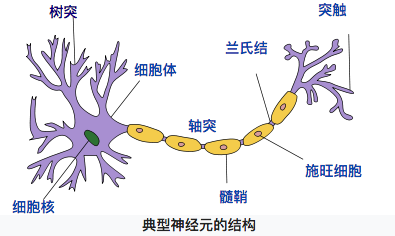【图1 典型神经元结构 （图片来自维基百科）】
神经元大致可以分为树突、突触、细胞体和轴突。树突为神经元的输入通道，其功能是将其它神经元的动作电位传递至细胞体。其它神经元的动作电位借由位于树突分支上的多个突触传递至树突上。神经细胞可以视为有两种状态的机器，激活时为“是”，不激活时为“否”。神经细胞的状态取决于从其他神经细胞接收到的信号量，以及突触的性质（抑制或加强）。当信号量超过某个阈值时，细胞体就会被激活，产生电脉冲。电脉冲沿着轴突并通过突触传递到其它神经元。（内容来自维基百科“感知机”）
同理，我们的神经元模型就是为了模拟上述过程，典型的神经元模型如下：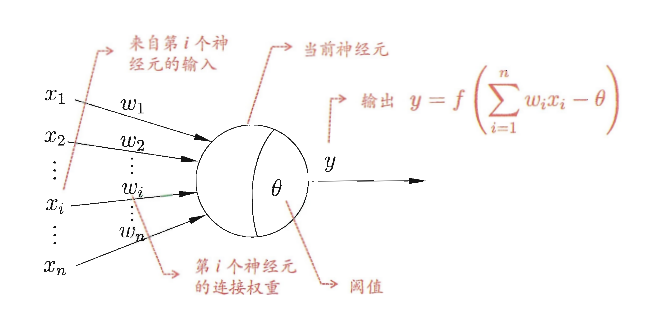【图2 典型神经元模型结构 （摘自周志华老师《机器学习》第97页）】
这个模型中，每个神经元都接受来自其它神经元的输入信号，每个信号都通过一个带有权重的连接传递，神经元把这些信号加起来得到一个总输入值，然后将总输入值与神经元的阈值进行对比（模拟阈值电位），然后通过一个“激活函数”处理得到最终的输出（模拟细胞的激活），这个输出又会作为之后神经元的输入一层一层传递下去。
1.2 神经元激活函数
本文主要介绍2种激活函数，分别是

s

i

g

m

o

i

d

sigmoid

和

r

e

l

u

relu

函数，函数公式如下：

s

i

g

m

o

i

d

(

z

)

=

1

1

+

e

−

z

sigmoid(z)=\frac{1}{1+e^{-z}}

r

e

l

u

(

z

)

=

{

z

z

&gt;

0

0

z

≤

0

relu(z)= \left\{ \begin{array}{rcl} z &amp; z&gt;0\\ 0&amp;z\leq0\end{array} \right.

做函数图如下：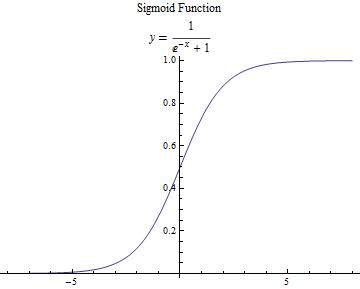s

i

g

m

o

i

d

(

z

)

sigmoid(z)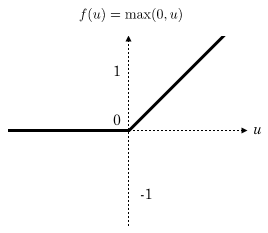r

e

l

u

(

z

)

relu(z)

【图3 激活函数】

补充说明 【补充说明的内容建议在看完后文的反向传播部分之后再回来阅读，我只是为了文章结构的统一把这部分内容添加在了这里】
引入激活函数的目的是在模型中引入非线性。如果没有激活函数，那么无论你的神经网络有多少层，最终都是一个线性映射，单纯的线性映射无法解决线性不可分问题。引入非线性可以让模型解决线性不可分问题。
一般来说，在神经网络的中间层更加建议使用

r

e

l

u

relu

函数，两个原因：

r

e

l

u

relu

函数计算简单，可以加快模型速度；由于反向传播过程中需要计算偏导数，通过求导可以得到

s

i

g

m

o

i

d

sigmoid

函数导数的最大值为0.25，如果使用

s

i

g

m

o

i

d

sigmoid

函数的话，每一层的反向传播都会使梯度最少变为原来的四分之一，当层数比较多的时候可能会造成梯度消失，从而模型无法收敛。

1.3 神经网络结构
我们使用如下神经网络结构来进行介绍，第0层是输入层（3个神经元）， 第1层是隐含层（2个神经元），第2层是输出层：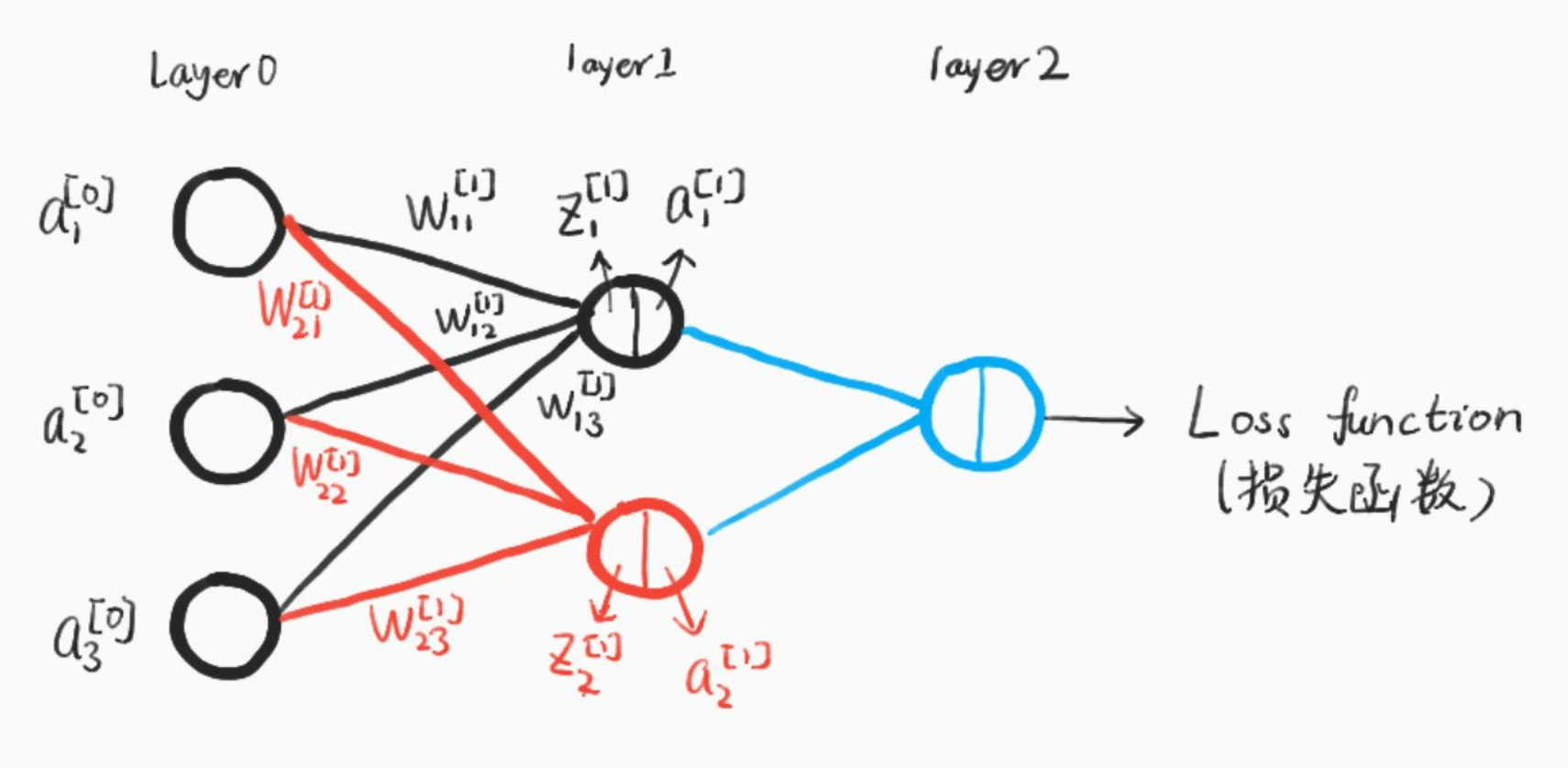【图4 神经网络结构（手绘）】
我们使用以下符号约定，

w

j

k

[

l

]

w_{jk}^{[l]}

表示从网络第

(

l

−

1

)

t

h

(l-1)^{th}

中

k

t

h

k^{th}

个神经元指向第

l

t

h

l^{th}

中第

j

t

h

j^{th}

个神经元的连接权重，比如上图中

w

21

[

1

]

w^{}_{21}

即从第0层第1个神经元指向第1层第2个神经元的权重。同理，我们使用

b

j

[

l

]

b^{[l]}_j

来表示第

l

t

h

l^{th}

层中第

j

t

h

j^{th}

神经元的偏差，用

z

j

[

l

]

z^{[l]}_j

来表示第

l

t

h

l^{th}

层中第

j

t

h

j^{th}

神经元的线性结果,用

a

j

[

l

]

a^{[l]}_j

来表示第

l

t

h

l^{th}

层中第

j

t

h

j^{th}

神经元的激活函数输出。
激活函数使用符号

σ

\sigma

表示，因此，第

l

t

h

l^{th}

层中第

j

t

h

j^{th}

神经元的激活为：

a

j

[

l

]

=

σ

(

∑

k

w

j

k

[

l

]

a

k

[

l

−

1

]

+

b

j

[

l

]

)

a^{[l]}_j=\sigma(\sum_kw^{[l]}_{jk}a^{[l-1]}_k+b^{[l]}_j)

现在，我们使用矩阵形式重写这个公式：
定义

w

[

l

]

w^{[l]}

表示权重矩阵，它的每一个元素表示一个权重，即每一行都是连接第

l

l

层的权重，用上图举个例子就是：

w

[

1

]

=

[

w

11

[

1

]

w

12

[

1

]

w

13

[

1

]

w

21

[

1

]

w

22

[

1

]

w

23

[

1

]

]

w^{}=\left[ \begin{array}{cc} w_{11}^{} &amp; w_{12}^{} &amp; w_{13}^{} \\ w_{21}^{}&amp; w_{22}^{} &amp; w_{23}^{}\end{array}\right]

同理，

b

[

1

]

=

[

b

1

[

1

]

b

2

[

1

]

]

b^{}=\left[ \begin{array}{cc}b^{}_1 \\ b^{}_2 \end{array}\right]

z

[

1

]

=

[

w

11

[

1

]

w

12

[

1

]

w

13

[

1

]

w

21

[

1

]

w

22

[

1

]

w

23

[

1

]

]

⋅

[

a

1

[

0

]

a

2

[

0

]

a

3

[

0

]

]

+

[

b

1

[

1

]

b

2

[

1

]

]

=

[

w

11

[

1

]

a

1

[

0

]

+

w

12

[

1

]

a

2

[

0

]

+

w

13

[

1

]

a

3

[

0

]

+

b

1

[

1

]

w

21

[

1

]

a

1

[

0

]

+

w

22

[

1

]

a

2

[

0

]

+

w

23

[

1

]

a

3

[

0

]

+

b

2

[

1

]

]

z^{}=\left[ \begin{array}{cc} w_{11}^{} &amp; w_{12}^{} &amp; w_{13}^{} \\ w_{21}^{}&amp; w_{22}^{} &amp; w_{23}^{}\end{array}\right]\cdot \left[ \begin{array}{cc} a^{}_1 \\ a^{}_2 \\ a^{}_3 \end{array}\right] +\left[ \begin{array}{cc}b^{}_1 \\ b^{}_2 \end{array}\right]=\left[ \begin{array}{cc} w_{11}^{}a^{}_1+w_{12}^{}a^{}_2+w_{13}^{}a^{}_3+b^{}_1 \\ w^{}_{21}a^{}_1+w_{22}^{}a^{}_2+w_{23}^{}a^{}_3+b^{}_2\end{array}\right]

更一般地，我们可以把前向传播过程表示：

a

[

l

]

=

σ

(

w

[

l

]

a

[

l

−

1

]

+

b

[

l

]

)

a^{[l]}=\sigma(w^{[l]}a^{[l-1]}+b^{[l]})

到这里，我们已经讲完了前向传播的过程，值得注意的是，这里我们只有一个输入样本，对于多个样本同时输入的情况是一样的，只不过我们的输入向量不再是一列，而是m列，每一个都表示一个输入样本。
多样本输入情况下的表示为：

Z

[

l

]

=

w

[

l

]

⋅

A

[

l

−

1

]

+

b

[

l

]

Z^{[l]}=w^{[l]}\cdot A^{[l-1]}+b^{[l]}

A

[

l

]

=

σ

(

Z

[

l

]

)

A^{[l]}=\sigma(Z^{[l]})

其中，此时

A

[

l

−

1

]

=

[

∣

∣

…

∣

a

[

l

−

1

]

(

1

)

a

[

l

−

1

]

(

2

)

…

a

[

l

−

1

]

(

m

)

∣

∣

…

∣

]

A^{[l-1]}=\left[ \begin{array}{cc} |&amp;|&amp;\ldots&amp;| \\a^{[l-1](1)}&amp;a^{[l-1](2)}&amp;\ldots&amp;a^{[l-1](m)} \\ |&amp;|&amp;\ldots&amp;|\end{array}\right]

每一列都表示一个样本，从样本1到m

w

[

l

]

w^{[l]}

的含义和原来完全一样，

Z

[

l

]

Z^{[l]}

也会变成m列，每一列表示一个样本的计算结果。

之后我们的叙述都是先讨论单个样本的情况，再扩展到多个样本同时计算。

2. 损失函数和代价函数
说实话，**损失函数（Loss Function）和代价函数（Cost Function）**并没有一个公认的区分标准，很多论文和教材似乎把二者当成了差不多的东西。
为了后面描述的方便，我们把二者稍微做一下区分（这里的区分仅仅对本文适用，对于其它的文章或教程需要根据上下文自行判断含义）：

损失函数主要指的是对于单个样本的损失或误差；代价函数表示多样本同时输入模型的时候总体的误差——每个样本误差的和然后取平均值。

举个例子，如果我们把单个样本的损失函数定义为：

L

(

a

,

y

)

=

−

[

y

⋅

l

o

g

(

a

)

+

(

1

−

y

)

⋅

l

o

g

(

1

−

a

)

]

L(a,y)=-[y \cdot log(a)+(1-y)\cdot log(1-a)]

那么对于m个样本，代价函数则是：

C

=

−

1

m

∑

i

=

0

m

(

y

(

i

)

⋅

l

o

g

(

a

(

i

)

)

+

(

1

−

y

(

i

)

)

⋅

l

o

g

(

1

−

a

(

i

)

)

)

C=-\frac{1}{m}\sum_{i=0}^m(y^{(i)}\cdot log(a^{(i)})+(1-y^{(i)})\cdot log(1-a^{(i)}))

3. 反向传播
反向传播的基本思想就是通过计算输出层与期望值之间的误差来调整网络参数，从而使得误差变小。
反向传播的思想很简单，然而人们认识到它的重要作用却经过了很长的时间。后向传播算法产生于1970年，但它的重要性一直到David Rumelhart，Geoffrey Hinton和Ronald Williams于1986年合著的论文发表才被重视。
事实上，人工神经网络的强大力量几乎就是建立在反向传播算法基础之上的。反向传播基于四个基础等式，数学是优美的，仅仅四个等式就可以概括神经网络的反向传播过程，然而理解这种优美可能需要付出一些脑力。事实上，反向传播如此之难，以至于相当一部分初学者很难进行独立推导。所以如果读者是初学者，希望读者可以耐心地研读本节。对于初学者，我觉得拿出1-3个小时来学习本小节是比较合适的，当然，对于熟练掌握反向传播原理的读者，你可以在十几分钟甚至几分钟之内快速浏览本节的内容。
3.1 矩阵补充知识
对于大部分理工科的研究生，以及学习过矩阵论或者工程矩阵理论相关课程的读者来说，可以跳过本节。
本节主要面向只学习过本科线性代数课程或者已经忘记矩阵论有关知识的读者。
总之，具备了本科线性代数知识的读者阅读这一小节应该不会有太大问题。本节主要在线性代数的基础上做一些扩展。（不排除少数本科线性代数课程也涉及到这些内容，如果感觉讲的简单的话，勿喷）
3.1.1 求梯度矩阵
假设函数

f

:

R

m

×

n

→

R

f:R^{m\times n}\rightarrow R

可以把输入矩阵（shape:

m

×

n

m\times n

）映射为一个实数。那么，函数

f

f

的梯度定义为：

∇

A

f

(

A

)

=

[

∂

f

(

A

)

∂

A

11

∂

f

(

A

)

∂

A

12

…

∂

f

(

A

)

∂

A

1

n

∂

f

(

A

)

∂

A

21

∂

f

(

A

)

∂

A

22

…

∂

f

(

A

)

∂

A

2

n

⋮

⋮

⋱

⋮

∂

f

(

A

)

∂

A

m

1

∂

f

(

A

)

∂

A

m

2

…

∂

f

(

A

)

∂

A

m

n

]

\nabla_Af(A)=\left[ \begin{array}{cc} \frac{\partial f(A)}{\partial A_{11}}&amp;\frac{\partial f(A)}{\partial A_{12}}&amp;\ldots&amp;\frac{\partial f(A)}{\partial A_{1n}} \\ \frac{\partial f(A)}{\partial A_{21}}&amp;\frac{\partial f(A)}{\partial A_{22}}&amp;\ldots&amp;\frac{\partial f(A)}{\partial A_{2n}} \\\vdots &amp;\vdots &amp;\ddots&amp;\vdots\\ \frac{\partial f(A)}{\partial A_{m1}}&amp;\frac{\partial f(A)}{\partial A_{m2}}&amp;\ldots&amp;\frac{\partial f(A)}{\partial A_{mn}}\end{array}\right]

即

(

∇

A

f

(

A

)

)

i

j

=

∂

f

(

A

)

∂

A

i

j

(\nabla_Af(A))_{ij}=\frac{\partial f(A)}{\partial A_{ij}}

同理，一个输入是向量（向量一般指列向量，本文在没有特殊声明的情况下默认指的是列向量）的函数

f

:

R

n

×

1

→

R

f:R^{n\times 1}\rightarrow R

，则有：

∇

x

f

(

x

)

=

[

∂

f

(

x

)

∂

x

1

∂

f

(

x

)

∂

x

2

⋮

∂

f

(

x

)

∂

x

n

]

\nabla_xf(x)=\left[ \begin{array}{cc}\frac{\partial f(x)}{\partial x_1}\\ \frac{\partial f(x)}{\partial x_2}\\ \vdots \\ \frac{\partial f(x)}{\partial x_n} \end{array}\right]

注意：这里涉及到的梯度求解的前提是函数

f

f

返回的是一个实数，如果函数返回的是一个矩阵或者向量，那么我们是没有办法求梯度的。比如，对函数

f

(

A

)

=

∑

i

=

0

m

∑

j

=

0

n

A

i

j

2

f(A)=\sum_{i=0}^m\sum_{j=0}^nA_{ij}^2

，由于返回一个实数,我们可以求解梯度矩阵。如果

f

(

x

)

=

A

x

(

A

∈

R

m

×

n

,

x

∈

R

n

×

1

)

f(x)=Ax (A\in R^{m\times n}, x\in R^{n\times 1})

，由于函数返回一个

m

m

行1列的向量，因此不能对

f

f

求梯度矩阵。

根据定义，很容易得到以下性质：

∇

x

(

f

(

x

)

+

g

(

x

)

)

=

∇

x

f

(

x

)

+

∇

x

g

(

x

)

\nabla_x(f(x)+g(x))=\nabla_xf(x)+\nabla_xg(x)

∇

(

t

f

(

x

)

)

=

t

∇

f

(

x

)

,

t

∈

R

\nabla(tf(x))=t\nabla f(x), t\in R

有了上述知识，我们来举个例子：

定义函数

f

:

R

m

→

R

,

f

(

z

)

=

z

T

z

f:R^m\rightarrow R, f(z)=z^Tz

,那么很容易得到

∇

z

f

(

z

)

=

2

z

\nabla_zf(z)=2z

，具体请读者自己证明。

3.1.2 海塞矩阵
定义一个输入为

n

n

维向量，输出为实数的函数

f

:

R

n

→

R

f:R^n\rightarrow R

，那么海塞矩阵（Hessian Matrix）定义为多元函数

f

f

的二阶偏导数构成的方阵：

∇

x

2

f

(

x

)

=

[

∂

2

f

(

x

)

∂

x

1

2

∂

2

f

(

x

)

∂

x

1

∂

x

2

…

∂

2

f

(

x

)

∂

x

1

∂

x

n

∂

2

f

(

x

)

∂

x

2

∂

x

1

∂

2

f

(

x

)

∂

x

2

2

…

∂

2

f

(

x

)

∂

x

2

∂

x

n

⋮

⋮

⋱

⋮

∂

2

f

(

x

)

∂

x

n

∂

x

1

∂

2

f

(

x

)

∂

x

n

∂

x

2

…

∂

2

f

(

x

)

∂

x

n

2

]

\nabla^2_xf(x)=\left[ \begin{array}{cc} \frac{\partial^2f(x)}{\partial x_1^2}&amp;\frac{\partial^2f(x)}{\partial x_1\partial x_2}&amp;\ldots &amp;\frac{\partial^2f(x)}{\partial x_1\partial x_n}\\ \frac{\partial^2f(x)}{\partial x_2\partial x_1}&amp;\frac{\partial^2f(x)}{\partial x_2^2}&amp;\ldots&amp;\frac{\partial^2f(x)}{\partial x_2\partial x_n}\\ \vdots&amp;\vdots&amp;\ddots&amp;\vdots\\\frac{\partial^2f(x)}{\partial x_n\partial x_1}&amp;\frac{\partial^2f(x)}{\partial x_n\partial x_2}&amp;\ldots&amp;\frac{\partial^2f(x)}{\partial x_n^2}\end{array}\right]

由上式可以看出，海塞矩阵总是对称阵。

注意：很多人把海塞矩阵看成

∇

x

f

(

x

)

\nabla _xf(x)

的导数，这是不对的。只能说，海塞矩阵的每个元素都是函数

f

f

二阶偏导数。那么，有什么区别呢？
首先，来看正确的解释。**海塞矩阵的每个元素是函数

f

f

的二阶偏导数。**拿

∂

2

f

(

x

)

∂

x

1

∂

x

2

\frac{\partial^2f(x)}{\partial x_1\partial x_2}

举个例子，函数

f

f

对

x

1

x_1

求偏导得到的是一个实数，比如

∂

2

f

(

x

)

∂

x

1

=

x

2

3

x

1

\frac{\partial^2f(x)}{\partial x_1}=x_2^3x_1

，因此继续求偏导是有意义的,继续对

x

2

x_2

求偏导可以得到

3

x

1

x

2

2

3x_1x_2^2

。
然后,来看一下错误的理解。把海塞矩阵看成

∇

x

f

(

x

)

\nabla _xf(x)

的导数，也就是说错误地以为

∇

x

2

f

(

x

)

=

∇

x

(

∇

x

f

(

x

)

)

\nabla^2_xf(x)=\nabla_x(\nabla_xf(x))

，要知道，

∇

x

f

(

x

)

\nabla_xf(x)

是一个向量，而在上一小节我们已经重点强调过，在我们的定义里对向量求偏导是没有定义的。
但是

∇

x

∂

f

(

x

)

∂

x

i

\nabla_x\frac{\partial f(x)}{\partial x_i}

是有意义的，因为

∂

f

(

x

)

∂

x

i

\frac{\partial f(x)}{\partial x_i}

是一个实数，具体地：

∇

x

∂

f

(

x

)

∂

x

i

=

[

∂

2

f

(

x

)

∂

x

i

∂

x

1

∂

2

f

(

x

)

∂

x

i

∂

x

2

⋮

∂

2

f

(

x

)

∂

x

i

∂

x

n

]

\nabla_x\frac{\partial f(x)}{\partial x_i}=\left[ \begin{array}{cc} \frac{\partial^2f(x)}{\partial x_i\partial x_1}\\\frac{\partial^2f(x)}{\partial x_i\partial x_2}\\\vdots\\\frac{\partial^2f(x)}{\partial x_i\partial x_n}\end{array}\right]

即海塞矩阵的第i行（或列）。
希望读者可以好好区分。

3.1.3 总结
根据3.1.1和3.1.2小节的内容很容易得到以下等式：

b

∈

R

n

,

x

∈

R

n

,

A

∈

R

n

×

n

并

且

A

是

对

称

矩

阵

b\in R^{n}, x\in R^n, A\in R^{n\times n}并且A 是对称矩阵

b

,

x

b,x

均为列向量   那么，

∇

x

b

T

x

=

b

\nabla_xb^Tx=b

∇

x

x

T

A

x

=

2

A

x

(

A

是

对

称

阵

)

\nabla_xx^TAx=2Ax(A是对称阵)

∇

x

2

x

T

A

x

=

2

A

(

A

是

对

称

阵

)

\nabla^2_xx^TAx=2A(A是对称阵)

这些公式可以根据前述定义自行推导，有兴趣的读者可以自己推导一下。 ####3.2 矩阵乘积和对应元素相乘   在下一节讲解反向传播原理的时候，尤其是把公式以矩阵形式表示的时候，需要大家时刻区分什么时候需要矩阵相乘，什么时候需要对应元素相乘。

比如对于矩阵

A

=

[

1

2

3

4

]

，

矩

阵

B

=

[

−

1

−

2

−

3

−

4

]

A=\left[ \begin{array}{cc} 1&amp;2\\3&amp;4\end{array}\right]，矩阵B=\left[ \begin{array}{cc} -1&amp;-2\\-3&amp;-4\end{array}\right]

矩阵相乘

A

B

=

[

1

×

−

1

+

2

×

−

3

1

×

−

2

+

2

×

−

4

3

×

−

1

+

4

×

−

3

3

×

−

2

+

4

×

−

4

]

=

[

−

7

−

10

−

15

−

22

]

AB=\left[\begin{array}{cc}1\times -1+2\times -3&amp;1\times -2+2\times -4\\3\times -1+4\times -3&amp;3\times -2+4\times -4\end{array}\right]=\left[\begin{array}{cc}-7&amp;-10\\-15&amp;-22\end{array}\right]

对应元素相乘使用符号

⊙

\odot

表示：

A

⊙

B

=

[

1

×

−

1

2

×

−

2

3

×

−

3

4

×

−

4

]

=

[

−

1

−

4

−

9

−

16

]

A\odot B=\left[\begin{array}{cc}1\times -1&amp;2\times -2 \\ 3\times -3&amp;4\times -4\end{array}\right]=\left[\begin{array}{cc}-1&amp;-4 \\ -9&amp;-16\end{array}\right]

3.3 梯度下降法原理
通过之前的介绍，相信大家都可以自己求解梯度矩阵（向量）了。
那么梯度矩阵（向量）求出来的意义是什么？从几何意义讲，梯度矩阵代表了函数增加最快的方向，因此，沿着与之相反的方向就可以更快找到最小值。如图5所示：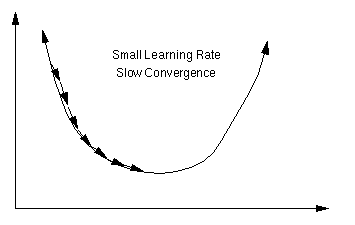【图5 梯度下降法 图片来自百度】   反向传播的过程就是利用梯度下降法原理，慢慢的找到代价函数的最小值，从而得到最终的模型参数。梯度下降法在反向传播中的具体应用见下一小节。
3.4 反向传播原理（四个基础等式）
反向传播能够知道如何更改网络中的权重

w

w

和偏差

b

b

来改变代价函数值。最终这意味着它能够计算偏导数

∂

L

(

a

[

l

]

,

y

)

∂

w

j

k

[

l

]

\frac{\partial L(a^{[l]},y)} {\partial w^{[l]}_{jk}}

和

∂

L

(

a

[

l

]

,

y

)

∂

b

j

[

l

]

\frac{\partial L(a^{[l]},y)}{\partial b^{[l]}_j}

为了计算这些偏导数，我们首先引入一个中间变量

δ

j

[

l

]

\delta^{[l]}_j

，我们把它叫做网络中第

l

t

h

l^{th}

层第

j

t

h

j^{th}

个神经元的误差。后向传播能够计算出误差

δ

j

[

l

]

\delta^{[l]}_j

，然后再将其对应回

∂

L

(

a

[

l

]

,

y

)

∂

w

j

k

[

l

]

\frac{\partial L(a^{[l]},y)}{\partial w^{[l]}_{jk}}

和

∂

L

(

a

[

l

]

,

y

)

∂

b

j

[

l

]

\frac{\partial L(a^{[l]},y)}{\partial b^{[l]}_j}

。
那么，如何定义每一层的误差呢？如果为第

l

l

层第

j

j

个神经元添加一个扰动

Δ

z

j

[

l

]

\Delta z^{[l]}_j

，使得损失函数或者代价函数变小，那么这就是一个好的扰动。通过选择

Δ

z

j

[

l

]

\Delta z^{[l]}_j

与

∂

L

(

a

[

l

]

,

y

)

∂

z

j

[

l

]

\frac{\partial L(a^{[l]}, y)}{\partial z^{[l]}_j}

符号相反（梯度下降法原理），就可以每次都添加一个好的扰动最终达到最优。
受此启发，我们定义网络层第

l

l

层中第

j

j

个神经元的误差为

δ

j

[

l

]

\delta^{[l]}_j

:

δ

j

[

l

]

=

∂

L

(

a

[

L

]

,

y

)

∂

z

j

[

l

]

\delta^{[l]}_j=\frac{\partial L(a^{[L], y})}{\partial z^{[l]}_j}

于是，每一层的误差向量可以表示为：

δ

[

l

]

=

[

δ

1

[

l

]

δ

2

[

l

]

⋮

δ

n

[

l

]

]

\delta ^{[l]}=\left[\begin{array}{cc}\delta ^{[l]}_1\\\delta ^{[l]}_2\\ \vdots \\ \delta ^{[l]}_n\end{array} \right]

下面开始正式介绍四个基础等式【确切的说是四组等式】

**注意：**这里我们的输入为单个样本(所以我们在下面的公式中使用的是损失函数而不是代价函数)。多个样本输入的公式会在介绍完单个样本后再介绍。

等式1 ：输出层误差

δ

j

[

L

]

=

∂

L

∂

a

j

[

L

]

σ

′

(

z

j

[

L

]

)

\delta^{[L]}_j=\frac{\partial L}{\partial a^{[L]}_j}\sigma^{&#x27;}(z^{[L]}_j)

其中，

L

L

表示输出层层数。以下用

∂

L

\partial L

表示

∂

L

(

a

[

L

]

,

y

)

\partial L(a^{[L]}, y)

写成矩阵形式是：

δ

[

L

]

=

∇

a

L

⊙

σ

′

(

z

[

L

]

)

\delta^{[L]}=\nabla _aL\odot \sigma^{&#x27;}(z^{[L]})

【注意是对应元素相乘，想想为什么？】

说明
根据本小节开始时的叙述，我们期望找到

∂

L

/

∂

z

j

[

l

]

\partial L \ /\partial z^{[l]}_j

，然后朝着方向相反的方向更新网络参数，并定义误差为：

δ

j

[

L

]

=

∂

L

∂

z

j

[

L

]

\delta^{[L]}_j=\frac{\partial L}{\partial z^{[L]}_j}

根据链式法则，

δ

j

[

L

]

=

∑

k

∂

L

∂

a

k

[

L

]

∂

a

k

[

L

]

∂

z

j

[

L

]

\delta^{[L]}_j = \sum_k \frac{\partial L}{\partial a^{[L]}_k} \frac{\partial a^{[L]}_k}{\partial z^{[L]}_j}

当

k

≠

j

k\neq j

时，

∂

a

k

[

L

]

/

∂

z

j

[

L

]

\partial a^{[L]}_k / \partial z^{[L]}_j

就为零。结果我们可以简化之前的等式为

δ

j

[

L

]

=

∂

L

∂

a

j

[

L

]

∂

a

j

[

L

]

∂

z

j

[

L

]

\delta^{[L]}_j = \frac{\partial L}{\partial a^{[L]}_j} \frac{\partial a^{[L]}_j}{\partial z^{[L]}_j}

重新拿出定义：

a

j

[

L

]

=

σ

(

z

j

[

L

]

)

a^{[L]}_j = \sigma(z^{[L]}_j)

，就可以得到：

δ

j

[

L

]

=

∂

L

∂

a

j

[

L

]

σ

′

(

z

j

[

L

]

)

\delta^{[L]}_j = \frac{\partial L}{\partial a^{[L]}_j} \sigma&#x27;(z^{[L]}_j)

再"堆砌"成向量形式就得到了我们的矩阵表示式（这也是为什么使用矩阵形式表示需要 对应元素相乘 的原因）。

等式2： 隐含层误差

δ

j

[

l

]

=

∑

k

w

k

j

[

l

+

1

]

δ

k

[

l

+

1

]

σ

′

(

z

j

[

l

]

)

\delta^{[l]}_j = \sum_k w^{[l+1]}_{kj} \delta^{[l+1]}_k \sigma&#x27;(z^{[l]}_j)

写成矩阵形式：

δ

[

l

]

=

[

w

[

l

+

1

]

T

δ

[

l

+

1

]

]

⊙

σ

′

(

z

[

l

]

)

\delta^{[l]}=[w^{[l+1]T}\delta^{[l+1]}]\odot \sigma ^{&#x27;}(z^{[l]})

说明：

z

k

[

l

+

1

]

=

∑

j

w

k

j

[

l

+

1

]

a

j

[

l

]

+

b

k

[

l

+

1

]

=

∑

j

w

k

j

[

l

+

1

]

σ

(

z

j

[

l

]

)

+

b

k

[

l

+

1

]

z^{[l+1]}_k=\sum_jw^{[l+1]}_{kj}a^{[l]}_j+b^{[l+1]}_k=\sum_jw^{[l+1]}_{kj}\sigma(z^{[l]}_j)+b^{[l+1]}_k

进行偏导可以获得：

∂

z

k

[

l

+

1

]

∂

z

j

[

l

]

=

w

k

j

[

l

+

1

]

σ

′

(

z

j

[

l

]

)

\frac{\partial z^{[l+1]}_k}{\partial z^{[l]}_j} = w^{[l+1]}_{kj} \sigma&#x27;(z^{[l]}_j)

代入得到：

δ

j

[

l

]

=

∑

k

w

k

j

[

l

+

1

]

δ

k

[

l

+

1

]

σ

′

(

z

j

[

l

]

)

\delta^{[l]}_j = \sum_k w^{[l+1]}_{kj} \delta^{[l+1]}_k \sigma&#x27;(z^{[l]}_j)

等式3：参数变化率

∂

L

∂

b

j

[

l

]

=

δ

j

[

l

]

\frac{\partial L}{\partial b^{[l]}_j}=\delta^{[l]}_j

∂

L

∂

w

j

k

[

l

]

=

a

k

[

l

−

1

]

δ

j

[

l

]

\frac{\partial L}{\partial w^{[l]}_{jk}}=a^{[l-1]}_k\delta^{[l]}_j

写成矩阵形式：

∂

L

∂

b

[

l

]

=

δ

[

l

]

\frac{\partial L}{\partial b^{[l]}}=\delta^{[l]}

∂

L

∂

w

[

l

]

=

δ

[

l

]

a

[

l

−

1

]

T

\frac{\partial L}{\partial w^{[l]}}=\delta^{[l]}a^{[l-1]T}

说明：
根据链式法则推导。   由于

z

j

[

l

]

=

∑

k

w

j

k

[

l

]

a

k

[

l

]

+

b

k

[

l

]

z^{[l]}_j=\sum_kw^{[l]}_{jk}a^{[l]}_k+b^{[l]}_k

对

b

j

[

l

]

b^{[l]}_j

求偏导得到：

∂

L

∂

b

j

[

l

]

=

∂

L

∂

z

j

[

l

]

∂

z

j

[

l

]

b

j

[

l

]

=

δ

j

[

l

]

\frac{\partial L}{\partial b^{[l]}_j}=\frac{\partial L}{\partial z^{[l]}_j}\frac{\partial z^{[l]}_j}{b^{[l]}_j}=\delta^{[l]}_j

对

w

j

k

[

l

]

w^{[l]}_{jk}

求偏导得到：

∂

L

∂

w

j

k

[

l

]

=

∂

L

∂

z

j

[

l

]

∂

z

j

[

l

]

w

j

k

[

l

]

=

a

k

[

l

−

1

]

δ

j

[

l

]

\frac{\partial L}{\partial w^{[l]}_{jk}}=\frac{\partial L}{\partial z^{[l]}_j}\frac{\partial z^{[l]}_j}{w^{[l]}_{jk}}=a^{[l-1]}_k\delta^{[l]}_j

最后再变成矩阵形式就好了。

对矩阵形式来说，需要特别注意维度的匹配。强烈建议读者在自己编写程序之前，先列出这些等式，然后仔细检查维度是否匹配。

很容易看出

∂

L

∂

w

[

l

]

\frac{\partial L}{\partial w^{[l]}}

是一个

d

i

m

(

δ

[

l

]

)

dim(\delta^{[l]})

行

d

i

m

(

a

[

l

−

1

]

)

dim(a^{[l-1]})

列的矩阵，和

w

[

l

]

w^{[l]}

的维度一致；

∂

L

∂

b

[

l

]

\frac{\partial L}{\partial b^{[l]}}

是一个维度为

d

i

m

(

δ

[

l

]

)

dim(\delta^{[l]})

的列向量

等式4：参数更新规则
这应该是这四组公式里最简单的一组了，根据梯度下降法原理，朝着梯度的反方向更新参数：

b

j

[

l

]

←

b

j

[

l

]

−

α

∂

L

∂

b

j

[

l

]

b^{[l]}_j\leftarrow b^{[l]}_j-\alpha \frac{\partial L}{\partial b^{[l]}_j}

w

j

k

[

l

]

←

w

j

k

[

l

]

−

α

∂

L

∂

w

j

k

[

l

]

w^{[l]}_{jk}\leftarrow w^{[l]}_{jk}-\alpha\frac{\partial L}{\partial w^{[l]}_{jk}}

写成矩阵形式：

b

[

l

]

←

b

[

l

]

−

α

∂

L

∂

b

[

l

]

b^{[l]}\leftarrow b^{[l]}-\alpha\frac{\partial L}{\partial b^{[l]}}

w

[

l

]

←

w

[

l

]

−

α

∂

L

∂

w

[

l

]

w^{[l]}\leftarrow w^{[l]}-\alpha\frac{\partial L}{\partial w^{[l]}}

这里的

α

\alpha

指的是学习率。学习率指定了反向传播过程中梯度下降的步长。

3.5 反向传播总结
我们可以得到如下最终公式：
3.5.1 单样本输入公式表
说明公式备注输出层误差

δ

[

L

]

=

∇

a

L

⊙

σ

′

(

z

[

L

]

)

\delta^{[L]}=\nabla _aL\odot \sigma^{&#x27;}(z^{[L]})

隐含层误差

δ

[

l

]

=

[

w

[

l

+

1

]

T

δ

[

l

+

1

]

]

⊙

σ

′

(

z

[

l

]

)

\delta^{[l]}=[w^{[l+1]T}\delta^{[l+1]}]\odot \sigma ^{&#x27;}(z^{[l]})

参数变化率

∂

L

∂

b

[

l

]

=

δ

[

l

]

\frac{\partial L}{\partial b^{[l]}}=\delta^{[l]}

∂

L

∂

w

[

l

]

=

δ

[

l

]

a

[

l

−

1

]

T

\frac{\partial L}{\partial w^{[l]}}=\delta^{[l]}a^{[l-1]T}

注意维度匹配参数更新

b

[

l

]

←

b

[

l

]

−

α

∂

L

∂

b

[

l

]

b^{[l]}\leftarrow b^{[l]}-\alpha\frac{\partial L}{\partial b^{[l]}}

w

[

l

]

←

w

[

l

]

−

α

∂

L

∂

w

[

l

]

w^{[l]}\leftarrow w^{[l]}-\alpha\frac{\partial L}{\partial w^{[l]}}

α

\alpha

是学习率
3.5.2 多样本输入公式表
多样本：需要使用代价函数，如果有m个样本，那么由于代价函数有一个

1

m

\frac{1}{m}

的常数项，因此所有的参数更新规则都需要有一个

1

m

\frac{1}{m}

的前缀。
多样本同时输入的时候需要格外注意维度匹配，一开始可能觉得有点混乱，但是不断加深理解就会豁然开朗。
说明公式备注输出层误差

d

Z

[

L

]

=

∇

A

C

⊙

σ

′

(

Z

[

L

]

)

dZ^{[L]}=\nabla _AC\odot \sigma^{&#x27;}(Z^{[L]})

此时

d

Z

[

l

]

dZ^{[l]}

不再是一个列向量，变成了一个

m

m

列的矩阵，每一列都对应一个样本的向量隐含层误差

d

Z

[

l

]

=

[

w

[

l

+

1

]

T

d

Z

[

l

+

1

]

]

⊙

σ

′

(

Z

[

l

]

)

dZ^{[l]}=[w^{[l+1]T}dZ^{[l+1]}]\odot \sigma ^{&#x27;}(Z^{[l]})

此时

d

Z

[

l

]

dZ^{[l]}

的维度是

n

×

m

n\times m

，

n

n

表示第l层神经元的个数，m表示样本数参数变化率

d

b

[

l

]

=

∂

C

∂

b

[

l

]

=

1

m

m

e

a

n

O

f

E

a

c

h

R

o

w

(

d

Z

[

l

]

)

d

w

[

l

]

=

∂

C

∂

w

[

l

]

=

1

m

d

Z

[

l

]

A

[

l

−

1

]

T

db^{[l]}=\frac{\partial C}{\partial b^{[l]}}=\frac{1}{m}meanOfEachRow(dZ^{[l]})\\dw^{[l]}=\frac{\partial C}{\partial w^{[l]}}=\frac{1}{m}dZ^{[l]}A^{[l-1]T}

更新

b

[

l

]

b^{[l]}

的时候需要对每行求均值； 注意维度匹配;

m

m

是样本个数参数更新

b

[

l

]

←

b

[

l

]

−

α

∂

C

∂

b

[

l

]

b^{[l]}\leftarrow b^{[l]}-\alpha\frac{\partial C}{\partial b^{[l]}}

w

[

l

]

←

w

[

l

]

−

α

∂

C

∂

w

[

l

]

w^{[l]}\leftarrow w^{[l]}-\alpha\frac{\partial C}{\partial w^{[l]}}

α

\alpha

是学习率
3.5.3 关于超参数
通过前面的介绍，相信读者可以发现BP神经网络模型有一些参数是需要设计者给出的，也有一些参数是模型自己求解的。
那么，哪些参数是需要模型设计者确定的呢？
比如，学习率

α

\alpha

，隐含层的层数，每个隐含层的神经元个数，激活函数的选取，损失函数（代价函数）的选取等等，这些参数被称之为超参数。
其它的参数，比如权重矩阵

w

w

和偏置系数

b

b

在确定了超参数之后是可以通过模型的计算来得到的，这些参数称之为普通参数，简称参数。
超参数的确定其实是很困难的。因为你很难知道什么样的超参数会让模型表现得更好。比如，学习率太小可能造成模型收敛速度过慢，学习率太大又可能造成模型不收敛；再比如，损失函数的设计，如果损失函数设计不好的话，可能会造成模型无法收敛；再比如，层数过多的时候，如何设计网络结构以避免梯度消失和梯度爆炸……
神经网络的程序比一般程序的调试难度大得多，因为它并不会显式报错，它只是无法得到你期望的结果，作为新手也很难确定到底哪里出了问题（对于自己设计的网络，这种现象尤甚，我目前也基本是新手，所以这些问题也在困扰着我）。当然，使用别人训练好的模型来微调看起来是一个捷径……
总之，神经网络至少在目前来看感觉还是黑箱的成分居多，希望通过大家的努力慢慢探索吧。
4. 是不是猫？
本小节主要使用上述公式来完成一个小例子，这个小小的神经网络可以告诉我们一张图片是不是猫。本例程参考了coursera的作业，有改动。
在实现代码之前，先把用到的公式列一个表格吧，这样对照着看大家更清晰一点(如果你没有2个显示器建议先把这些公式抄写到纸上，以便和代码对照)：
编号公式备注1

Z

[

l

]

=

w

[

l

]

A

[

l

−

1

]

+

b

[

l

]

Z^{[l]}=w^{[l]}A^{[l-1]}+b^{[l]}

2

A

[

l

]

=

σ

(

Z

[

l

]

)

A^{[l]}=\sigma(Z^{[l]})

3

d

Z

[

L

]

=

∇

A

C

⊙

σ

′

(

Z

[

L

]

)

dZ^{[L]}=\nabla_AC\odot\sigma^{&#x27;}(Z^{[L]})

4

d

Z

[

l

]

=

[

w

[

l

+

1

]

T

d

Z

[

l

+

1

]

]

⊙

σ

′

(

Z

[

l

]

)

dZ^{[l]}=[w^{[l+1]T}dZ^{[l+1]}]\odot \sigma ^{&#x27;}(Z^{[l]})

5

d

b

[

l

]

=

∂

C

∂

b

[

l

]

=

1

m

m

e

a

n

O

f

E

a

c

h

R

o

w

(

d

Z

[

l

]

)

db^{[l]}=\frac{\partial C}{\partial b^{[l]}}=\frac{1}{m}meanOfEachRow(dZ^{[l]})

6

d

w

[

l

]

=

∂

C

∂

w

[

l

]

=

1

m

d

Z

[

l

]

A

[

l

−

1

]

T

dw^{[l]}=\frac{\partial C}{\partial w^{[l]}}=\frac{1}{m}dZ^{[l]}A^{[l-1]T}

7

b

[

l

]

←

b

[

l

]

−

α

⋅

d

b

[

l

]

b^{[l]}\leftarrow b^{[l]}-\alpha \cdot db^{[l]}

8

w

[

l

]

←

w

[

l

]

−

α

⋅

d

w

[

l

]

w^{[l]}\leftarrow w^{[l]}-\alpha\cdot dw^{[l]}

9

d

A

[

l

]

=

w

[

l

]

T

⊙

d

Z

[

l

]

dA^{[l]}=w^{[l]T}\odot dZ^{[l]}

准备工作做的差不多了，让我们开始吧？等等，好像我们还没有定义代价函数是什么？OMG！好吧，看来我们得先把这个做好再继续了。
那先看结果吧，我们的代价函数是：

C

=

−

1

m

∑

i

=

1

m

(

y

(

i

)

l

o

g

(

a

[

L

]

(

i

)

)

+

(

1

−

y

(

i

)

)

l

o

g

(

1

−

a

[

L

]

(

i

)

)

)

C =-\frac{1}{m} \sum^{m}_{i=1}(y^{(i)}log(a^{[L](i)})+(1-y^{(i)})log(1-a^{[L](i)}))

其中，

m

m

是样本数量；
下面简单介绍一下这个代价函数是怎么来的（作者非数学专业，不严谨的地方望海涵）。 .   代价函数的确定用到了统计学中的**“极大似然法”**，既然这样，那就不可避免地要介绍一下“极大似然法”了。极大似然法简单来说就是“在模型已定，参数未知的情况下，根据结果估计模型中参数的一种方法"，换句话说，极大似然法提供了一种给定观察数据来评估模型参数的方法。
举个例子（本例参考了知乎相关回答），一个不透明的罐子里有黑白两种球（球仅仅颜色不同，大小重量等参数都一样）。有放回地随机拿出一个小球，记录颜色。重复10次之后发现7次是黑球，3次是白球。问你罐子里白球的比例？
相信很多人可以一口回答“30%”，那么，为什么呢？背后的原理是什么呢？
这里我们把每次取出一个球叫做一次抽样，把“抽样10次，7次黑球，3次白球”这个事件发生的概率记为

P

(

事

件

结

果

∣

M

o

d

e

l

)

P(事件结果|Model)

，我们的Model需要一个参数

p

p

表示白球的比例。那么

P

(

事

件

结

果

∣

M

o

d

e

l

)

=

p

3

(

1

−

p

)

7

P(事件结果|Model)=p^3(1-p)^7

。
好了，现在我们已经有事件结果的概率公式了，接下来求解模型参数

p

p

，根据极大似然法的思想，既然这个事件发生了，那么为什么不让这个事件（抽样10次，7次黑球，3次白球）发生的概率最大呢？因为显然概率大的事件发生才是合理的。于是就变成了求解

p

3

(

1

−

p

)

7

p^3(1-p)^7

取最大值的

p

p

，即导数为0，经过求导：

d

(

p

3

(

1

−

p

)

7

)

=

3

p

2

(

1

−

p

)

7

−

7

p

3

(

1

−

p

)

6

=

p

2

(

1

−

p

)

6

(

3

−

10

p

)

=

0

d(p^3(1-p)^7)=3p^2(1-p)^7-7p^3(1-p)^6=p^2(1-p)^6(3-10p)=0

求解可得

p

=

0.3

p=0.3

极大似然法有一个重要的假设：

假设所有样本独立同分布！！！

好了，现在来看看我们的神经网络模型。
最后一层我们用sigmoid函数求出一个激活输出a，如果a大于0.5，就表示这个图片是猫（

y

=

1

y=1

），否则就不是猫（

y

=

0

y=0

）。因此:

P

(

y

=

1

∣

x

;

θ

)

=

a

P(y=1|x;\theta)=a

P

(

y

=

0

∣

x

;

θ

)

=

1

−

a

P(y=0|x;\theta)=1-a

公式解释： 上述第一个公式表示，给定模型参数

θ

\theta

和输入

x

x

，是猫的概率是

P

(

y

=

1

∣

x

;

θ

)

=

a

P(y=1|x;\theta)=a

把两个公式合并成一个公式，即

p

(

y

∣

x

;

θ

)

=

a

y

(

1

−

a

)

(

1

−

y

)

p(y|x;\theta)=a^y(1-a)^{(1-y)}

这里的

θ

\theta

指的就是我们神经网络的权值参数和偏置参数。

那么似然函数

L

(

θ

)

=

p

(

Y

∣

X

;

θ

)

=

∏

i

=

1

m

p

(

y

(

i

)

∣

x

(

i

)

;

θ

)

=

∏

i

=

1

m

(

a

[

L

]

(

i

)

)

y

(

i

)

(

1

−

a

[

L

]

(

i

)

)

(

1

−

y

(

i

)

)

L(\theta)=p(Y|X;\theta)=\prod^m_{i=1}p(y^{(i)}|x^{(i)};\theta)=\prod^m_{i=1}(a^{[L](i)})^{y^{(i)}}(1-a^{[L](i)})^{(1-y^{(i)})}

变成对数形式：

l

o

g

(

L

(

θ

)

)

=

∑

i

=

1

m

(

y

(

i

)

l

o

g

(

a

[

L

]

(

i

)

)

+

(

1

−

y

(

i

)

)

l

o

g

(

1

−

a

[

L

]

(

i

)

)

)

log(L(\theta))=\sum^m_{i=1}(y^{(i)}log(a^{[L](i)})+(1-y^{(i)})log(1-a^{[L](i)}))

所以我们的目标就是最大化这个对数似然函数，也就是最小化我们的代价函数：

C

=

−

1

m

∑

i

=

1

m

(

y

(

i

)

l

o

g

(

a

[

L

]

(

i

)

)

+

(

1

−

y

(

i

)

)

l

o

g

(

1

−

a

[

L

]

(

i

)

)

)

C =-\frac{1}{m} \sum^{m}_{i=1}(y^{(i)}log(a^{[L](i)})+(1-y^{(i)})log(1-a^{[L](i)}))

其中，

m

m

是样本数量；
好了，终于可以开始写代码了，码字手都有点酸了，不得不说公式真的好难打。

由于代码比较简单就没有上传github。本文代码和数据文件可以在这里下载: https://pan.baidu.com/s/1q_PzaCSXOhRLOJVF5-vy2Q，密码： d7vx

4.1 辅助函数
辅助函数主要包括激活函数以及激活函数的反向传播过程函数： 其中，激活函数反向传播代码对应公式4和9.
def sigmoid(z):
"""
使用numpy实现sigmoid函数

参数：
Z numpy array
输出：
A 激活值（维数和Z完全相同）
"""
return 1/(1 + np.exp(-z))

def relu(z):
"""
线性修正函数relu

参数：
z numpy array
输出：
A 激活值（维数和Z完全相同）

"""
return np.array(z>0)*z

def sigmoidBackward(dA, cacheA):
"""
sigmoid的反向传播

参数：
dA 同层激活值
cacheA 同层线性输出
输出：
dZ 梯度

"""
s = sigmoid(cacheA)
diff = s*(1 - s)
dZ = dA * diff
return dZ

def reluBackward(dA, cacheA):
"""
relu的反向传播

参数：
dA 同层激活值
cacheA 同层线性输出
输出：
dZ 梯度

"""
Z = cacheA
dZ = np.array(dA, copy=True)
dZ[Z <= 0] = 0
return dZ

另外一个重要的辅助函数是数据读取函数和参数初始化函数：
def loadData(dataDir):
"""
导入数据

参数：
输出：
训练集，测试集以及标签
"""
train_set_x_orig = np.array(train_dataset["train_set_x"][:]) # your train set features
train_set_y_orig = np.array(train_dataset["train_set_y"][:]) # your train set labels

test_set_x_orig = np.array(test_dataset["test_set_x"][:]) # your test set features
test_set_y_orig = np.array(test_dataset["test_set_y"][:]) # your test set labels

classes = np.array(test_dataset["list_classes"][:]) # the list of classes

train_set_y_orig = train_set_y_orig.reshape((1, train_set_y_orig.shape))
test_set_y_orig = test_set_y_orig.reshape((1, test_set_y_orig.shape))

return train_set_x_orig, train_set_y_orig, test_set_x_orig, test_set_y_orig, classes

def iniPara(laydims):
"""
随机初始化网络参数

参数：
laydims 一个python list
输出：
parameters 随机初始化的参数字典（”W1“，”b1“，”W2“，”b2“, ...）
"""
np.random.seed(1)
parameters = {}
for i in range(1, len(laydims)):
parameters['W'+str(i)] = np.random.randn(laydims[i], laydims[i-1])/ np.sqrt(laydims[i-1])
parameters['b'+str(i)] = np.zeros((laydims[i], 1))
return parameters

4.2 前向传播过程

对应公式1和2.

def forwardLinear(W, b, A_prev):
"""
前向传播
"""
Z = np.dot(W, A_prev) + b
cache = (W, A_prev, b)
return Z, cache

def forwardLinearActivation(W, b, A_prev, activation):
"""
带激活函数的前向传播
"""
Z, cacheL = forwardLinear(W, b, A_prev)
cacheA = Z
if activation == 'sigmoid':
A = sigmoid(Z)
if activation == 'relu':
A = relu(Z)
cache = (cacheL, cacheA)
return A, cache

def forwardModel(X, parameters):
"""
完整的前向传播过程
"""
layerdim = len(parameters)//2
caches = []
A_prev = X
for i in range(1, layerdim):
A_prev, cache = forwardLinearActivation(parameters['W'+str(i)], parameters['b'+str(i)], A_prev, 'relu')
caches.append(cache)

AL, cache = forwardLinearActivation(parameters['W'+str(layerdim)], parameters['b'+str(layerdim)], A_prev, 'sigmoid')
caches.append(cache)

return AL, caches

4.3 反向传播过程

线性部分反向传播对应公式5和6。

def linearBackward(dZ, cache):
"""
线性部分的反向传播

参数：
dZ 当前层误差
cache （W, A_prev, b）元组
输出：
dA_prev 上一层激活的梯度
dW 当前层W的梯度
db 当前层b的梯度
"""
W, A_prev, b = cache
m = A_prev.shape

dW = 1/m*np.dot(dZ, A_prev.T)
db = 1/m*np.sum(dZ, axis = 1, keepdims=True)
dA_prev = np.dot(W.T, dZ)

return dA_prev, dW, db


非线性部分对应公式3、4、5和6 。

def linearActivationBackward(dA, cache, activation):
"""
非线性部分的反向传播

参数：
dA 当前层激活输出的梯度
cache （W, A_prev, b）元组
activation 激活函数类型
输出：
dA_prev 上一层激活的梯度
dW 当前层W的梯度
db 当前层b的梯度
"""
cacheL, cacheA = cache

if activation == 'relu':
dZ = reluBackward(dA, cacheA)
dA_prev, dW, db = linearBackward(dZ, cacheL)
elif activation == 'sigmoid':
dZ = sigmoidBackward(dA, cacheA)
dA_prev, dW, db = linearBackward(dZ, cacheL)

return dA_prev, dW, db


完整反向传播模型：

def backwardModel(AL, Y, caches):
"""
完整的反向传播过程

参数：
AL 输出层结果
Y 标签值
caches 【cacheL, cacheA】
输出：
diffs 梯度字典
"""
layerdim = len(caches)
Y = Y.reshape(AL.shape)
L = layerdim

diffs = {}

dAL = - (np.divide(Y, AL) - np.divide(1 - Y, 1 - AL))

currentCache = caches[L-1]
dA_prev, dW, db =  linearActivationBackward(dAL, currentCache, 'sigmoid')
diffs['dA' + str(L)], diffs['dW'+str(L)], diffs['db'+str(L)] = dA_prev, dW, db

for l in reversed(range(L-1)):
currentCache = caches[l]
dA_prev, dW, db =  linearActivationBackward(dA_prev, currentCache, 'relu')
diffs['dA' + str(l+1)], diffs['dW'+str(l+1)], diffs['db'+str(l+1)] = dA_prev, dW, db

return diffs

4.4 测试结果
打开你的jupyter notebook，运行我们的BP.ipynb文件，首先导入依赖库和数据集，然后使用一个循环来确定最佳的迭代次数大约为2000：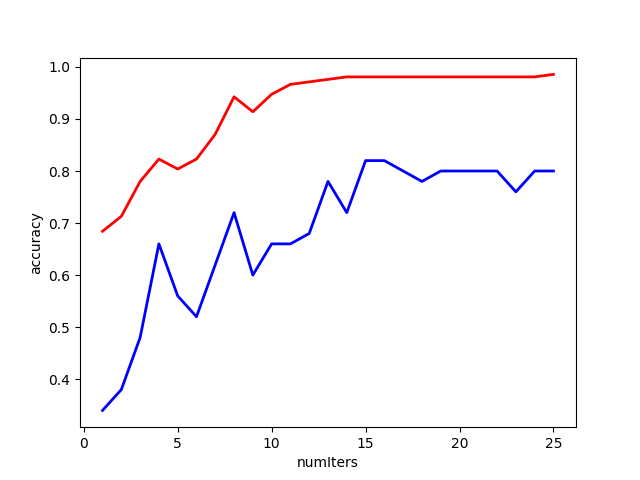【图6】
最后用一个例子来看一下模型的效果——判断一张图片是不是猫：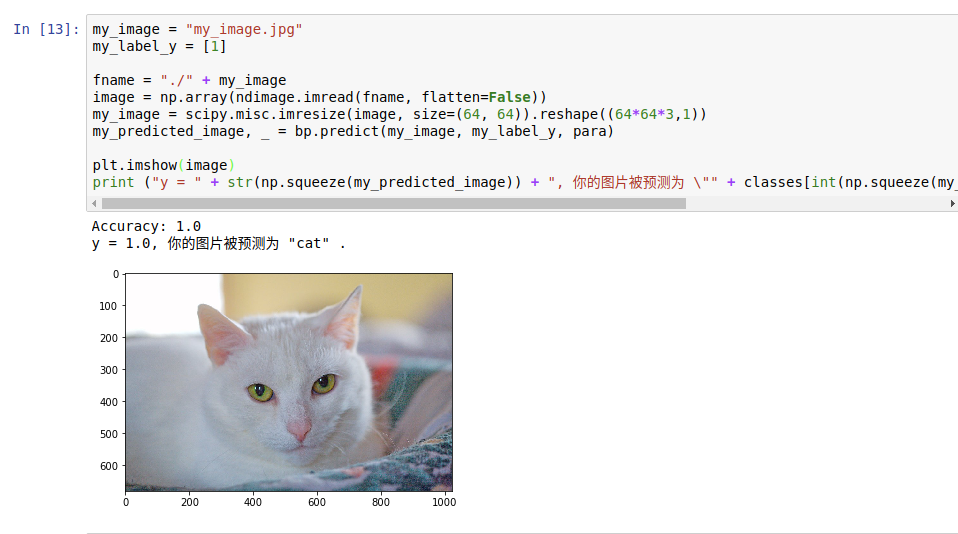【图7】
好了，测试到此结束。你也可以自己尝试其它的神经网络结构和测试其它图片。
5. 本文小结
本文主要叙述了经典的全连接神经网络结构以及前向传播和反向传播的过程。通过本文的学习，读者应该可以独立推导全连接神经网络的传播过程，对算法的细节烂熟于心。另外，由于本文里的公式大部分是我自己推导的，瑕疵之处，希望读者不吝赐教。
虽然这篇文章实现的例子并没有什么实际应用场景，但是自己推导一下这些数学公式并用代码实现对理解神经网络内部的原理很有帮助，继这篇博客之后，我还计划写一个如何自己推导并实现卷积神经网络的教程，如果有人感兴趣，请继续关注我！
本次内容就到这里，谢谢大家。
订正与答疑：
前向传播过程比较简单，我就不再赘述了。
这里主要针对反向传播过程中可能会出现的问题做一个总结：
1. 具体解释一下公式1里面的“堆砌”是什么意思？

δ

j

[

L

]

=

∑

k

∂

L

∂

a

k

[

L

]

∂

a

k

[

L

]

∂

z

j

[

L

]

\delta^{[L]}_j = \sum_k \frac{\partial L}{\partial a^{[L]}_k} \frac{\partial a^{[L]}_k}{\partial z^{[L]}_j}

有读者对这里不太理解，这其实是因为，我们的输出层不一定是只有一个神经元，可能有好多个神经元，因此损失函数是每个输出神经元“误差”之和，因此才会出现这种

∑

\sum

的形式，然后每个输出神经元的误差函数与其它神经元没有关系，所以只有

k

=

j

k=j

的时候值不是0.
另外，这里说的“堆砌”指的就是：

δ

[

l

]

=

[

∂

L

∂

a

1

[

L

]

∂

L

∂

a

2

[

L

]

⋮

]

⊙

[

σ

′

(

z

1

[

L

]

)

σ

′

(

z

2

[

L

]

)

⋮

]

\delta^{[l]}=\left[ \begin{array}{cc} \frac{\partial L}{\partial a^{[L]}_1} \\ \frac{\partial L}{\partial a^{[L]}_2} \\\vdots \end{array}\right]\odot\left[\begin{array}{cc}\sigma^{&#x27;}(z^{[L]}_1) \\\sigma^{&#x27;}(z^{[L]}_2)\\\vdots\end{array}\right]